Idea of what [[category theory]] is. Basic definition of mathematical concepts.

Notions that are important in category theory. Commutative diagrams; categories as kinds of [[context]]. At the end, how this fits in a bigger picture.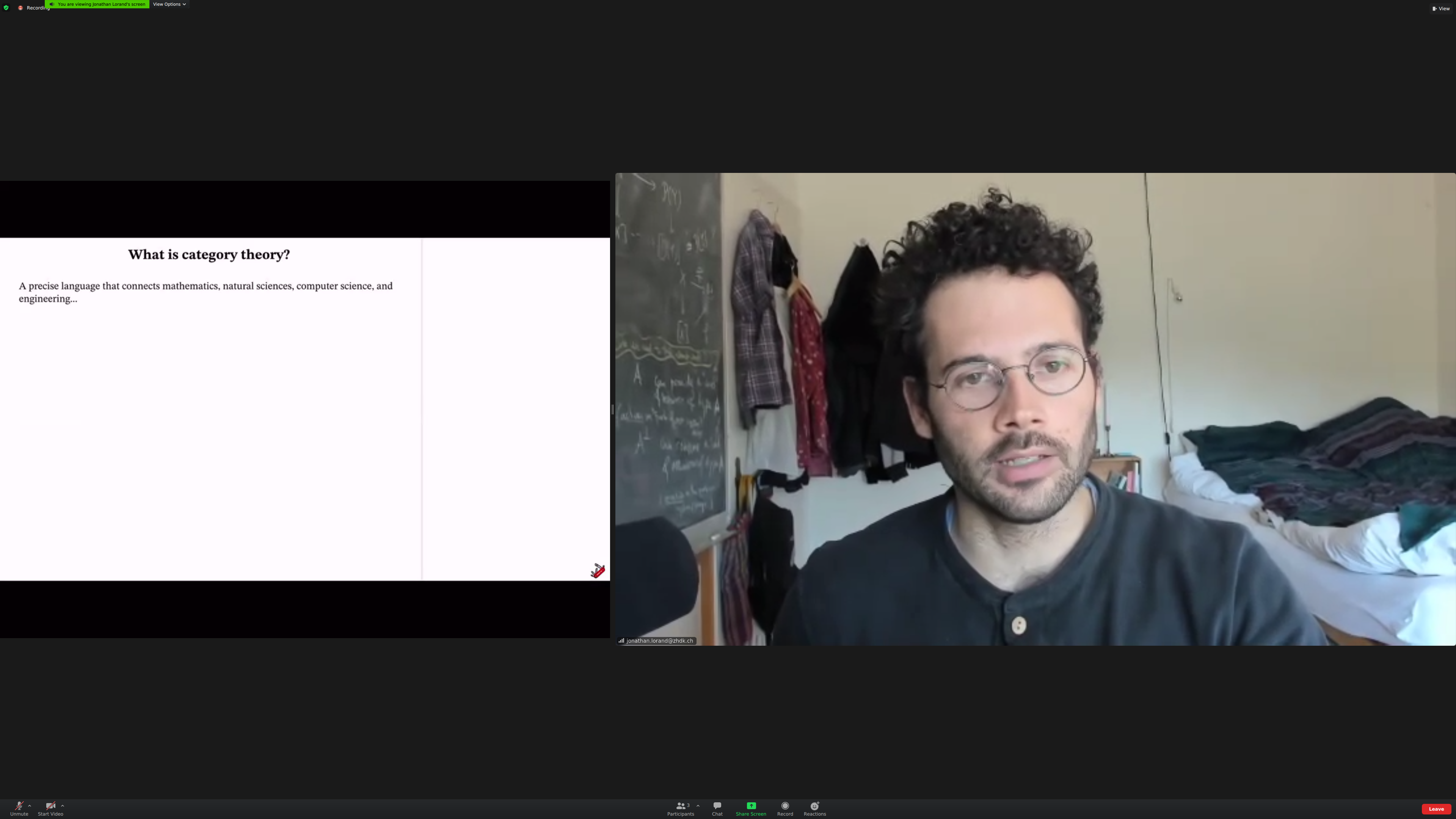Aside: I'm reading Feynman and this fits in amazingly well with the introduction of that book, six easy pieces.

An [[elegant framework]] for reasoning about situations where we are composing stuff together. [[modeling]], [[computation]].

Category theory is about [[composing stuff]] together.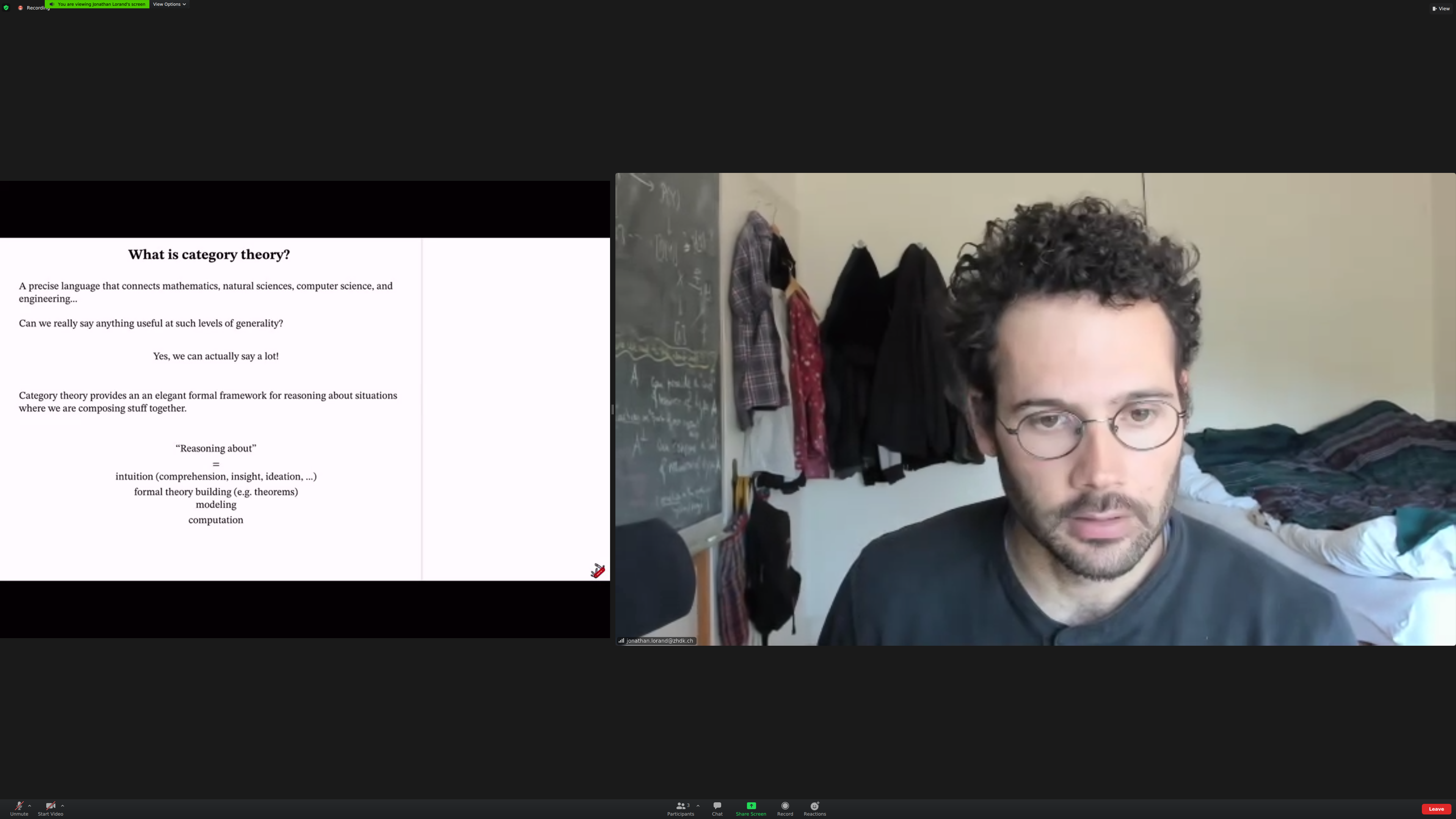Example: composing [[journeys]].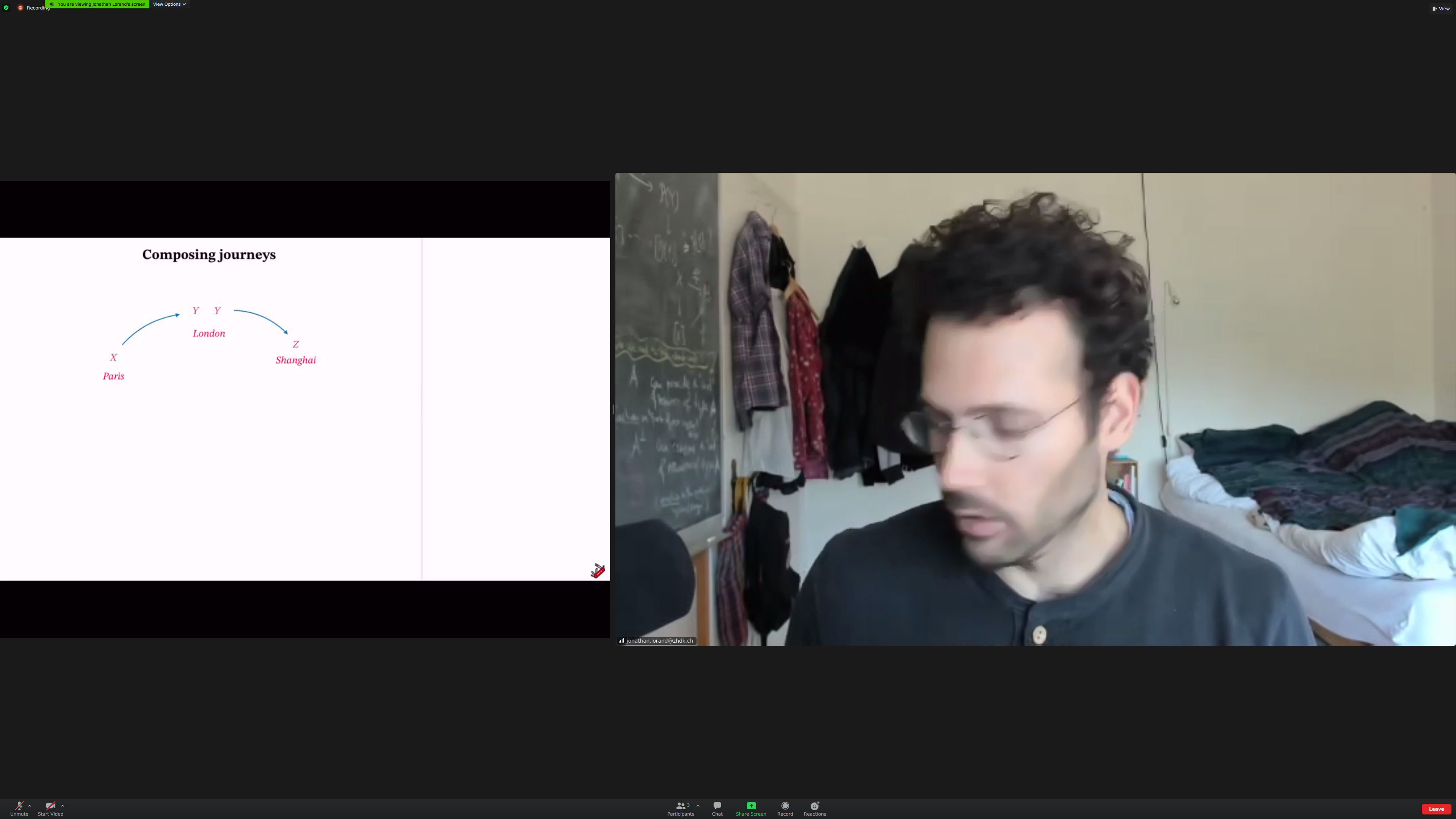Example: composing processes. Mathematical functions, algorithms, physical processes.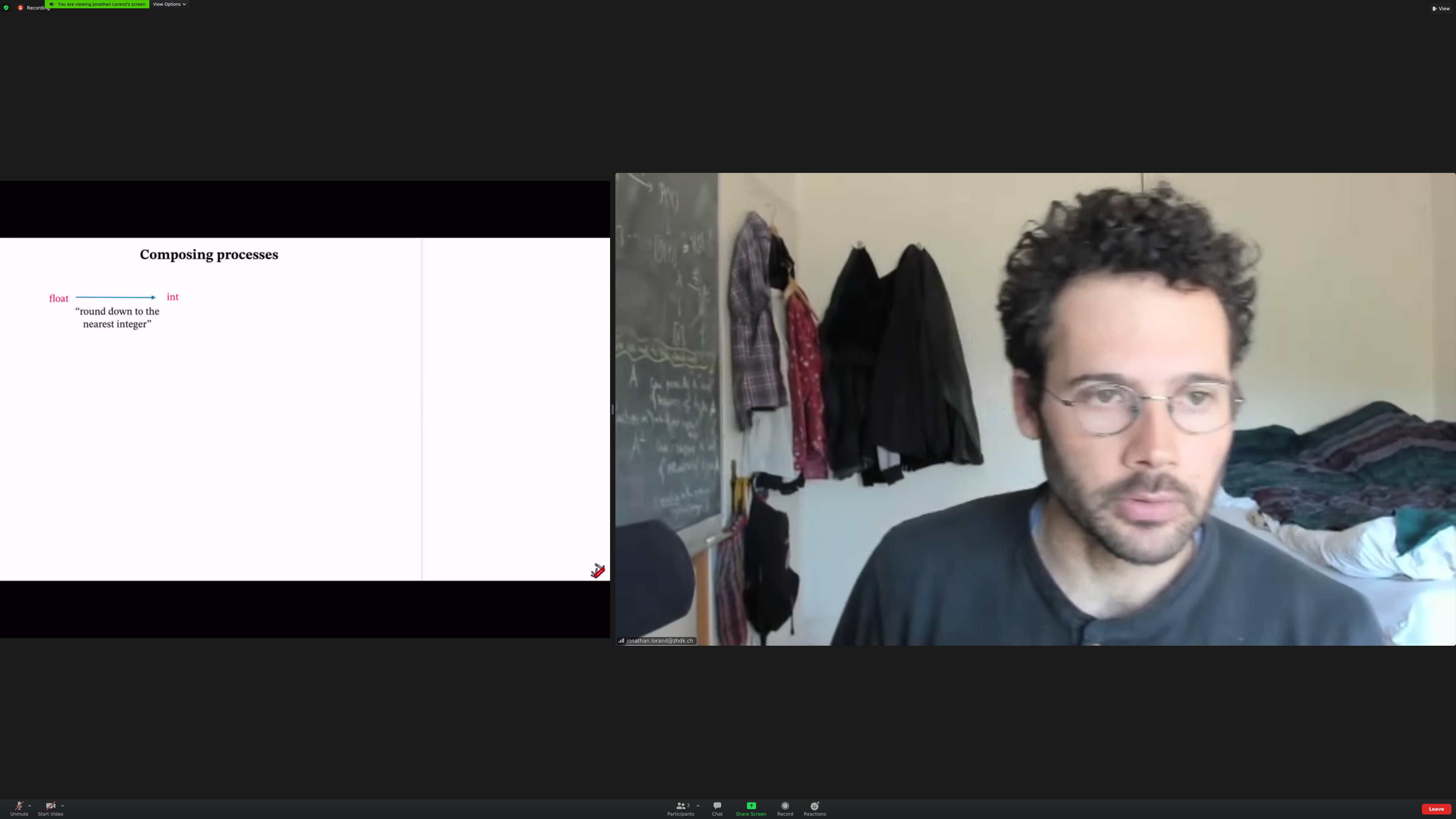Notation: boxes instead of arrows. Directionality is from left to right.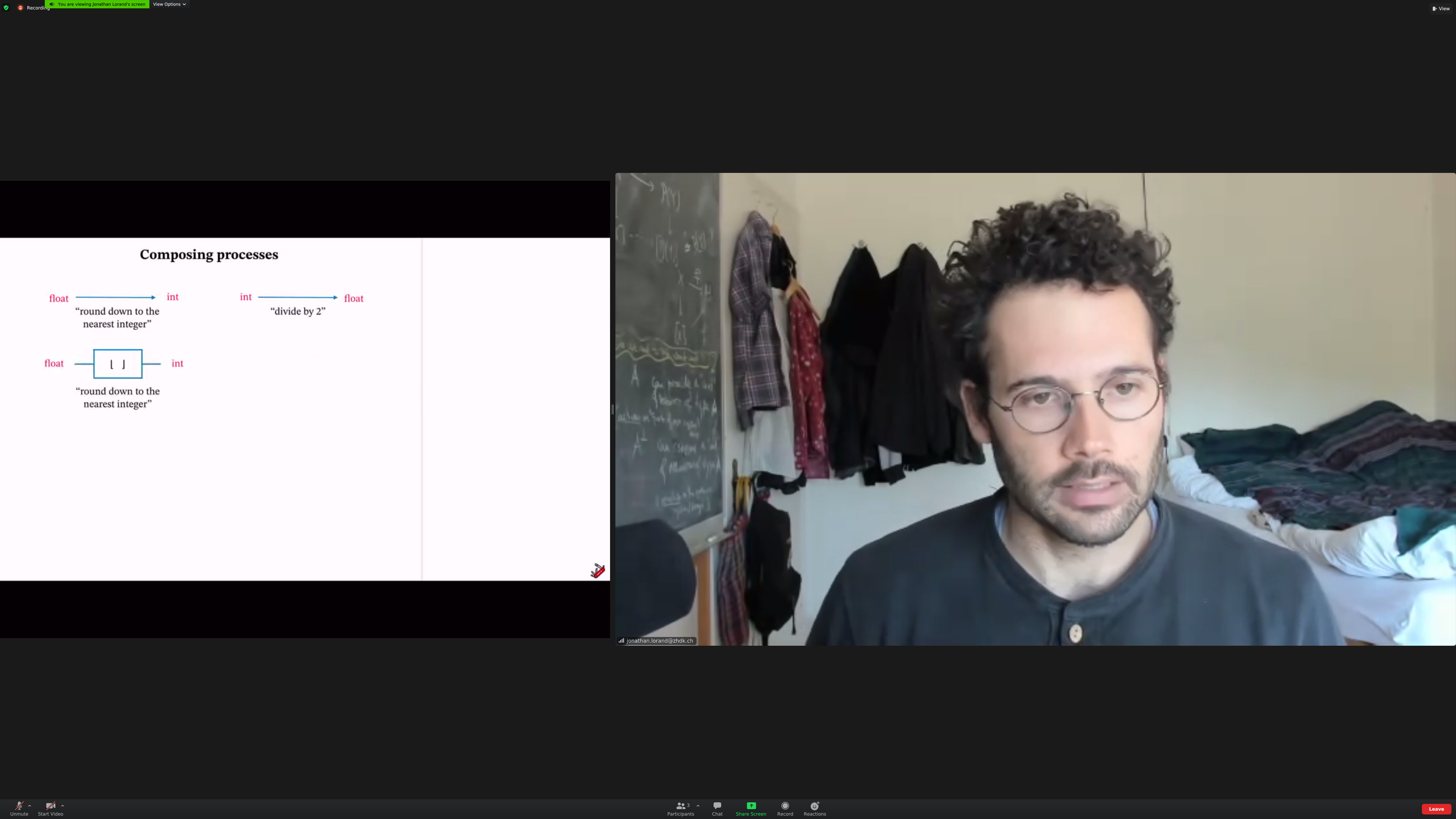The output of the first box is the same [[type]] as the input of the second.You can also compose physical components, of course.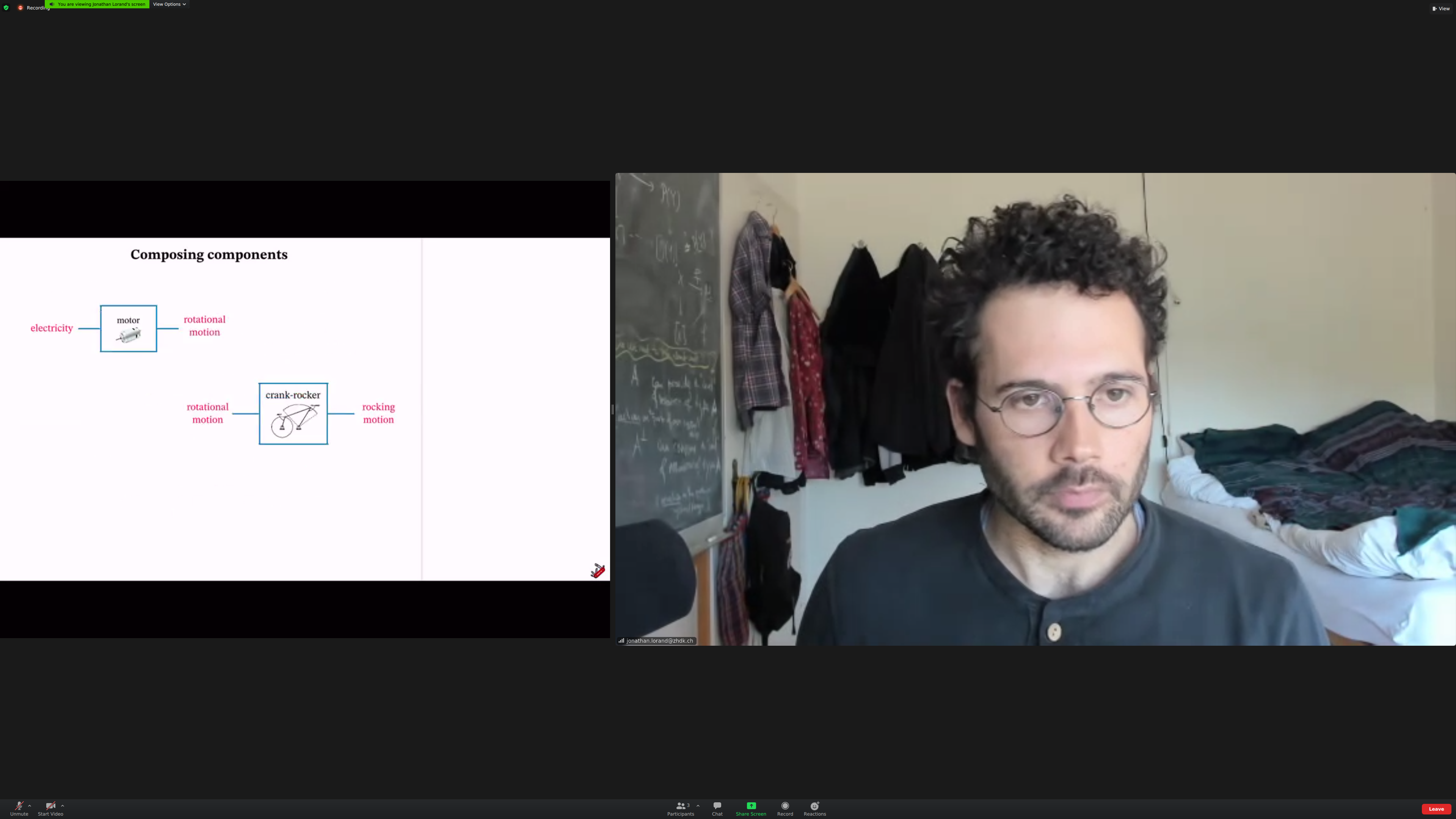And physical processes.The fundamental example is mathematical; composing functions as sets.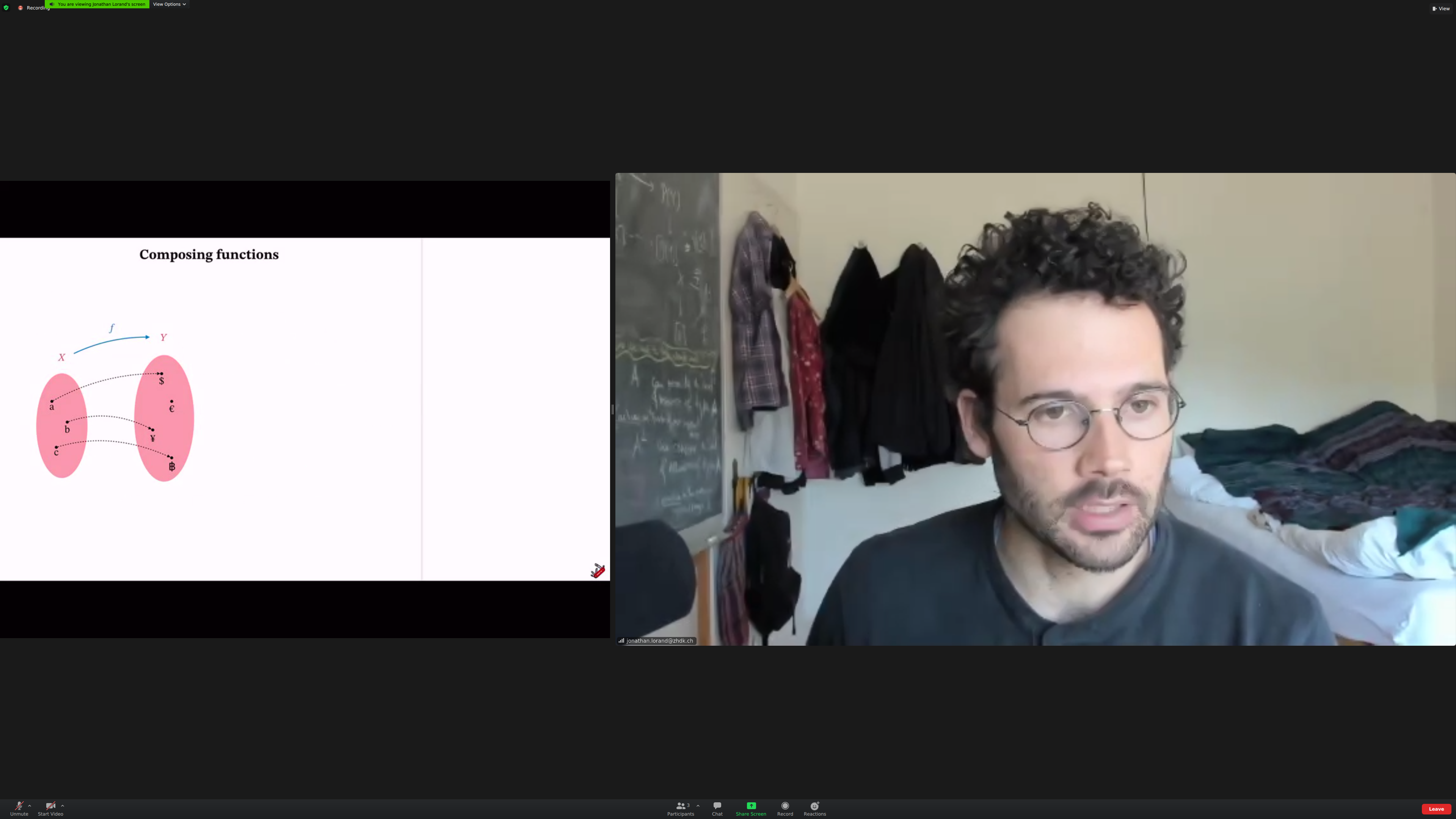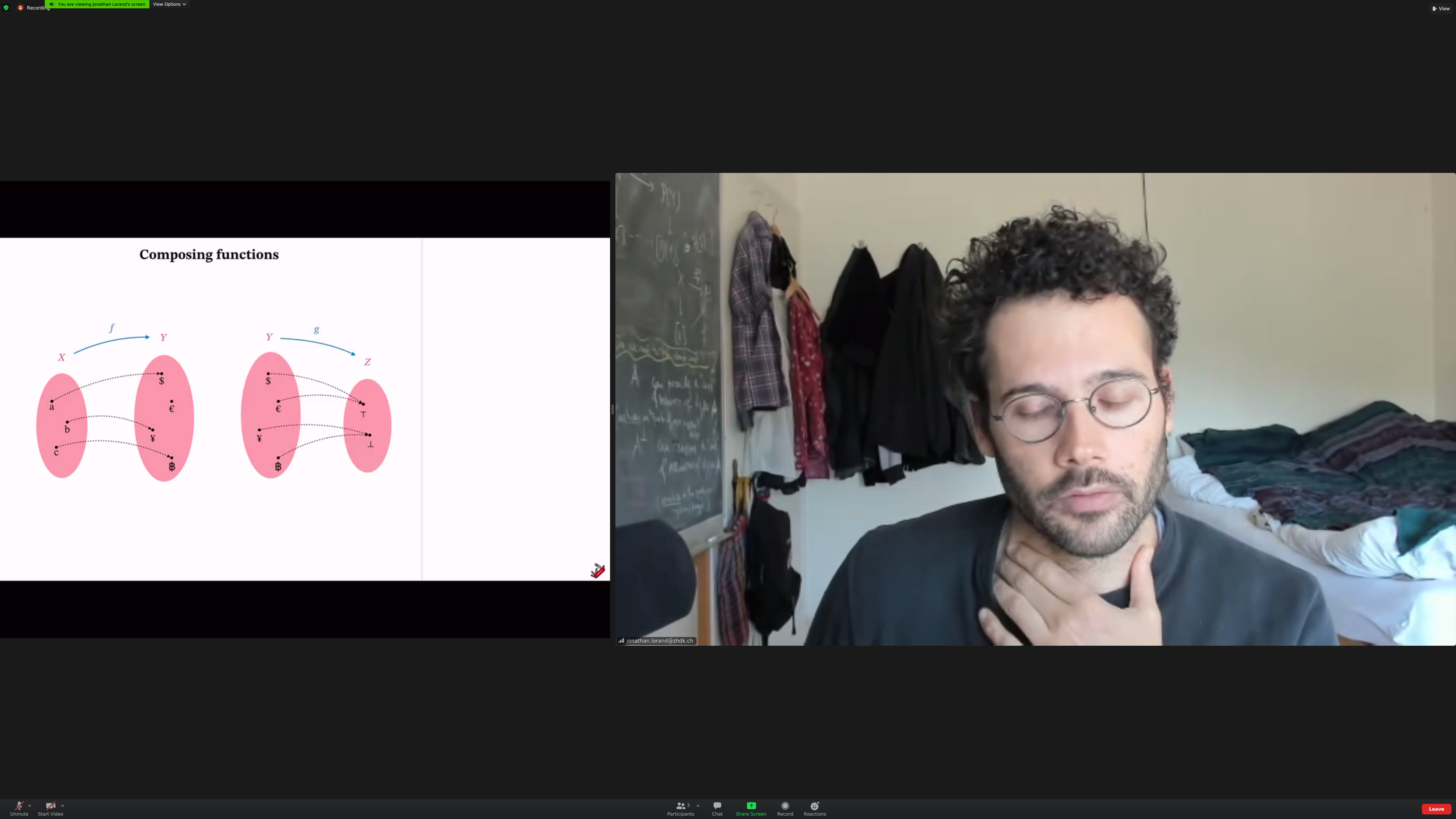Aside: we should put this on youtube.

We'll be used 'then' notations for compositions, not traditional.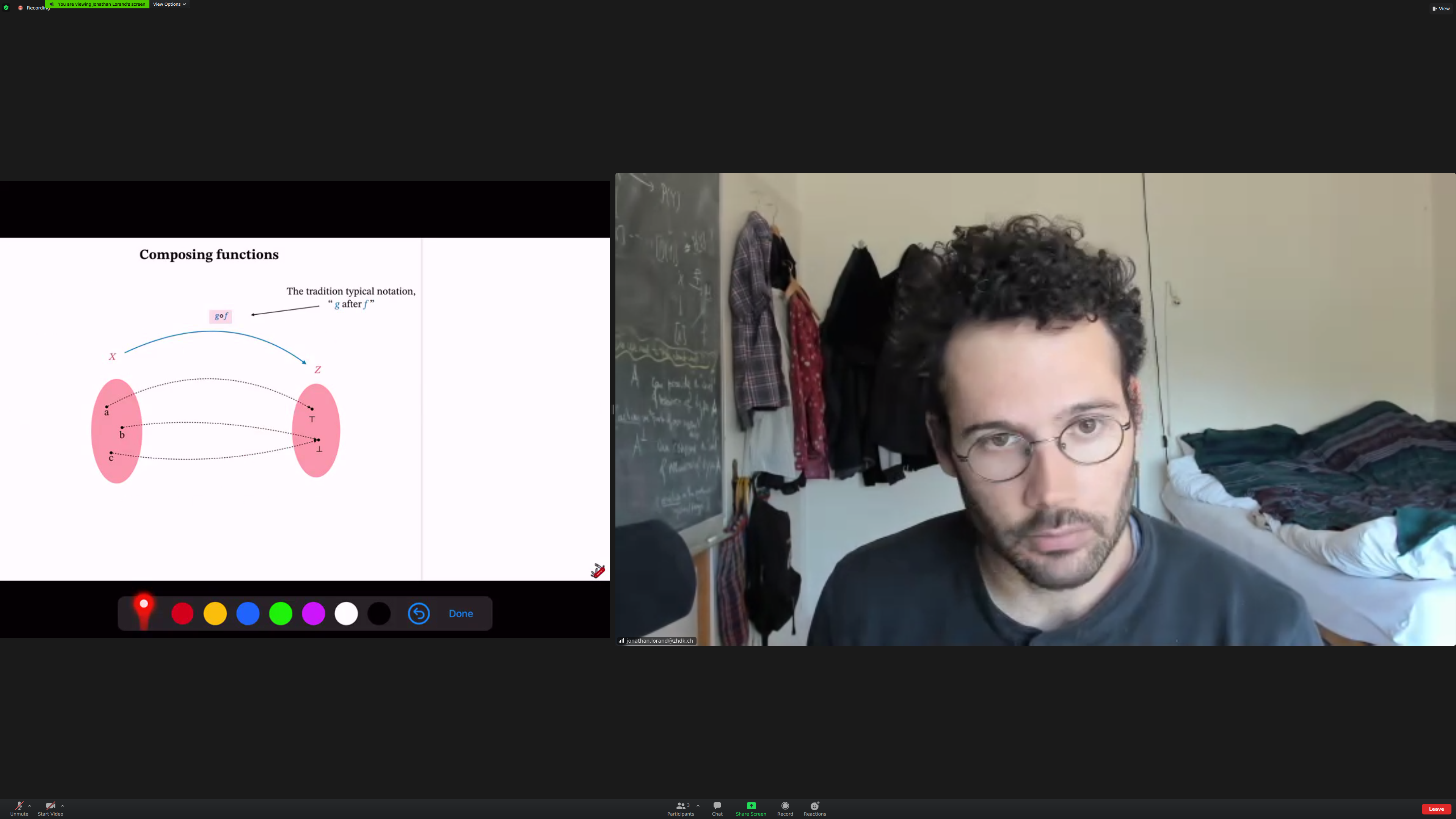We'll relate [[objects]]. The things doing the relating are [[morphisms]].

(here there should be a better screenshot, I couldn't get it on time)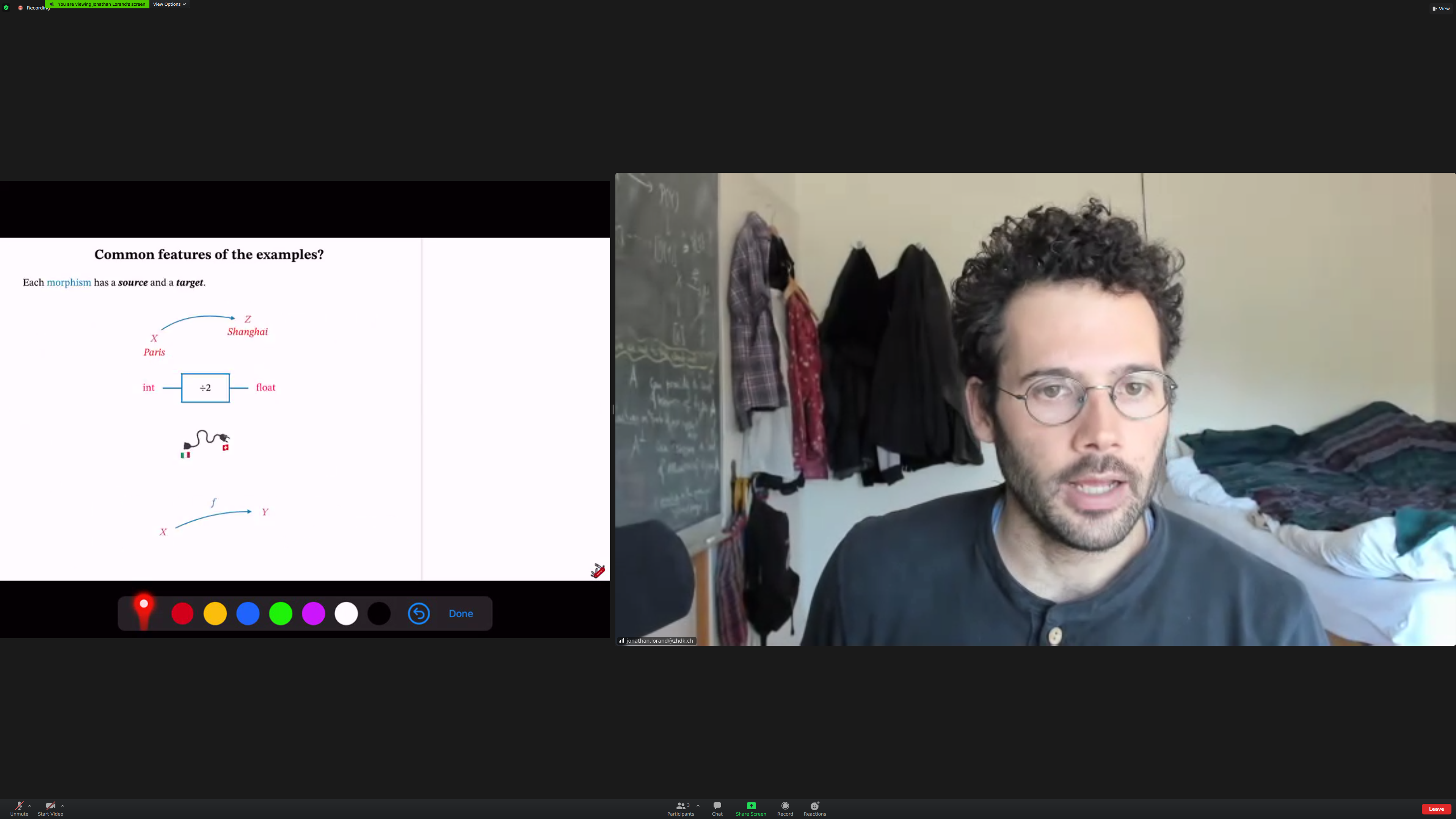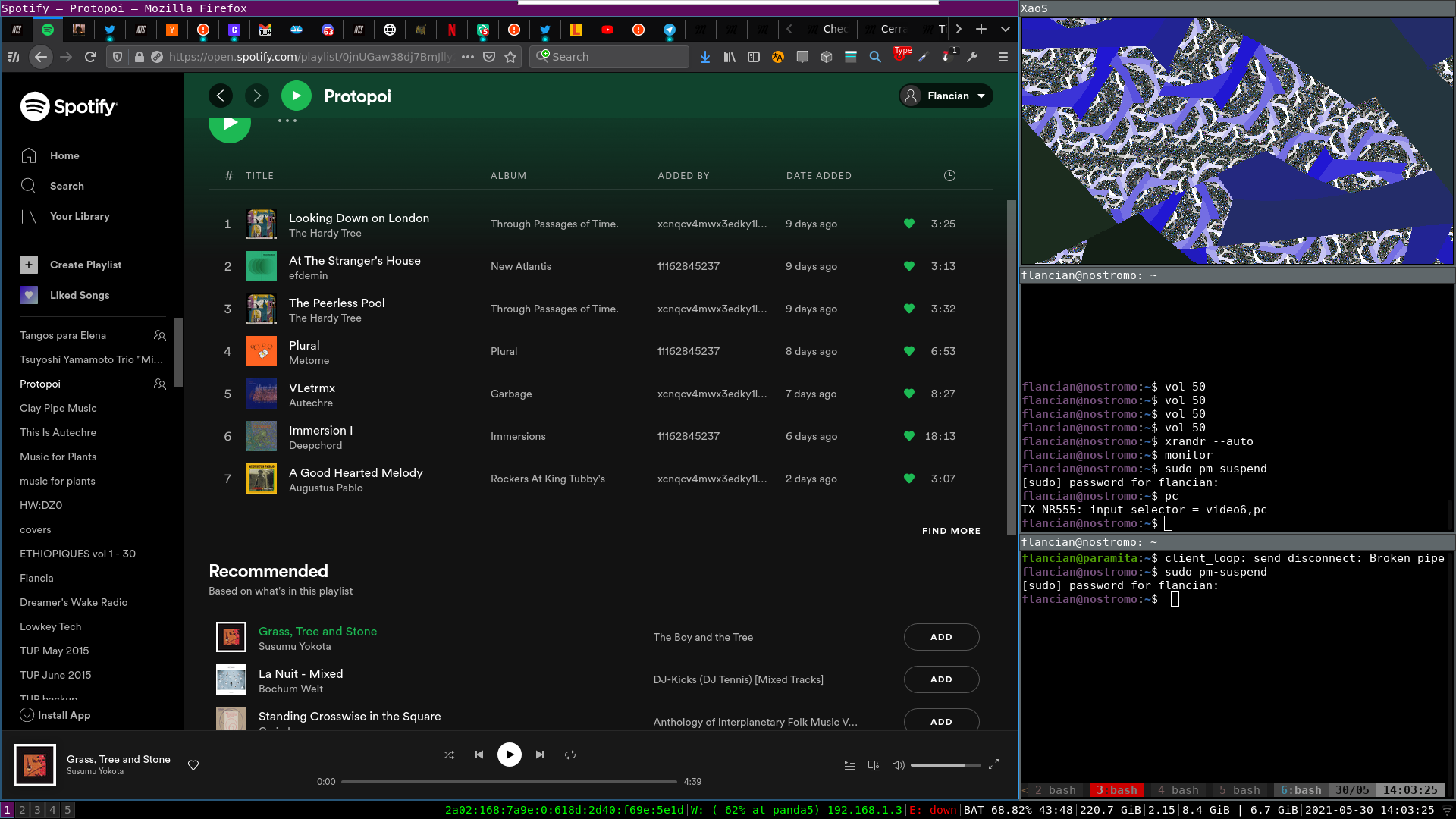Each morphism has a [[source]] and a [[target]].

We can compose morphisms when the target of the first matches the source of the second.

Common feature of all examples: they are [[associative]]. The order of composition doesn't matter; the order of application may. (check)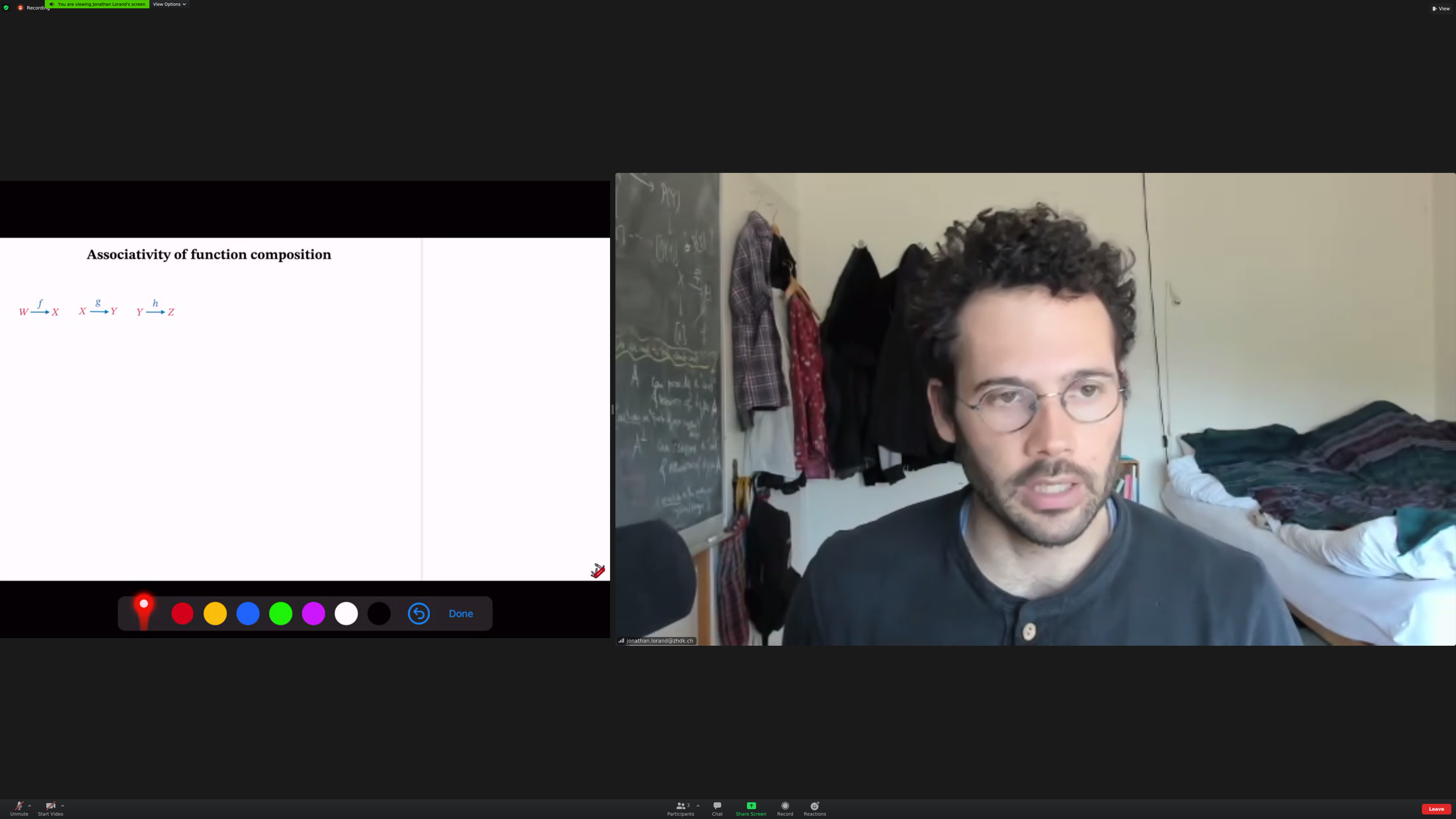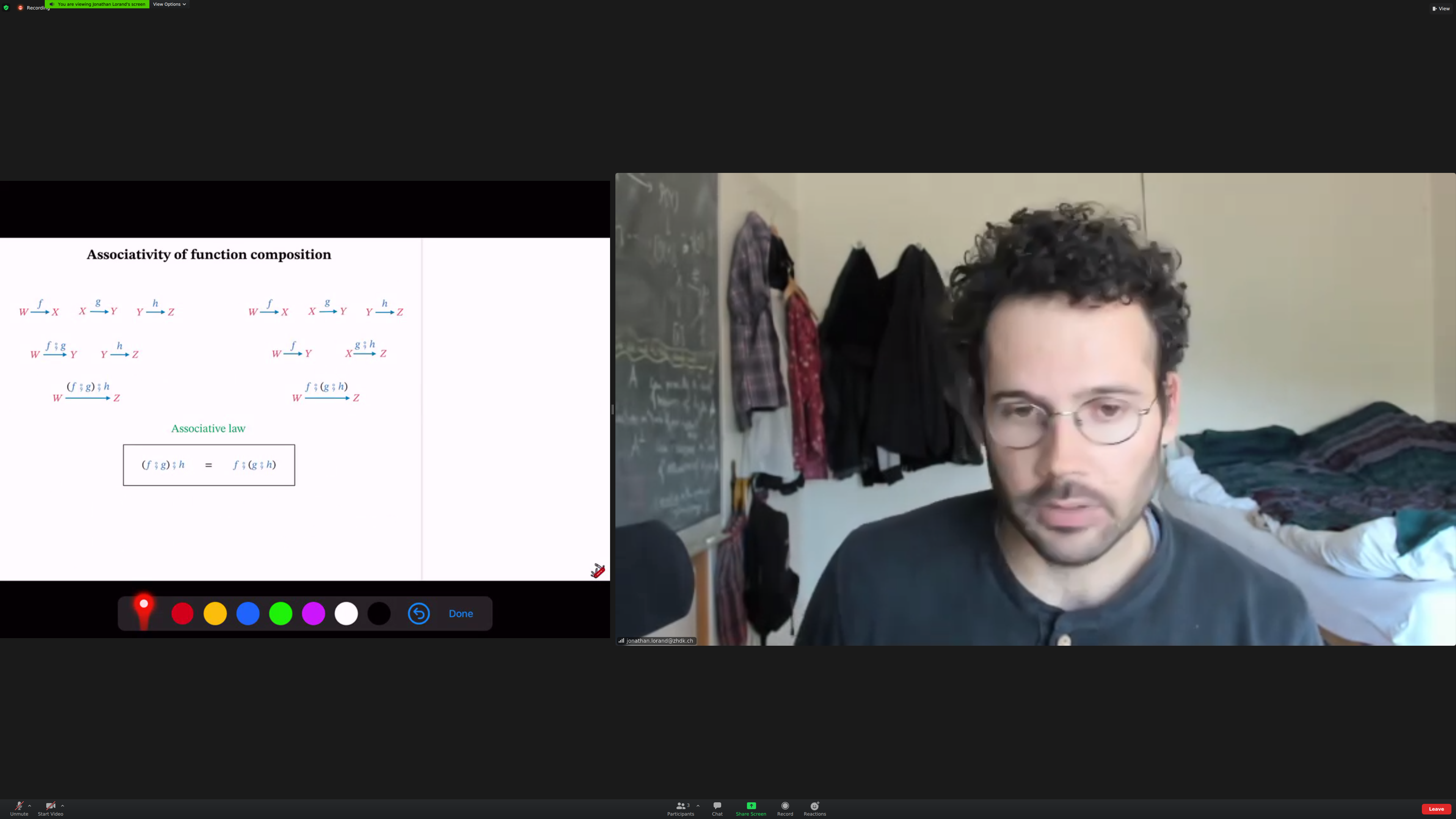This was [[true]] for all the examples we looked at so far.

Associativity means that brackets are not needed.Aside: going from a class in real time to screenshots is one morphism; going from screenshots to notes is another. Going from notes to a chapter or blog post is another.

Associativiy in plugs: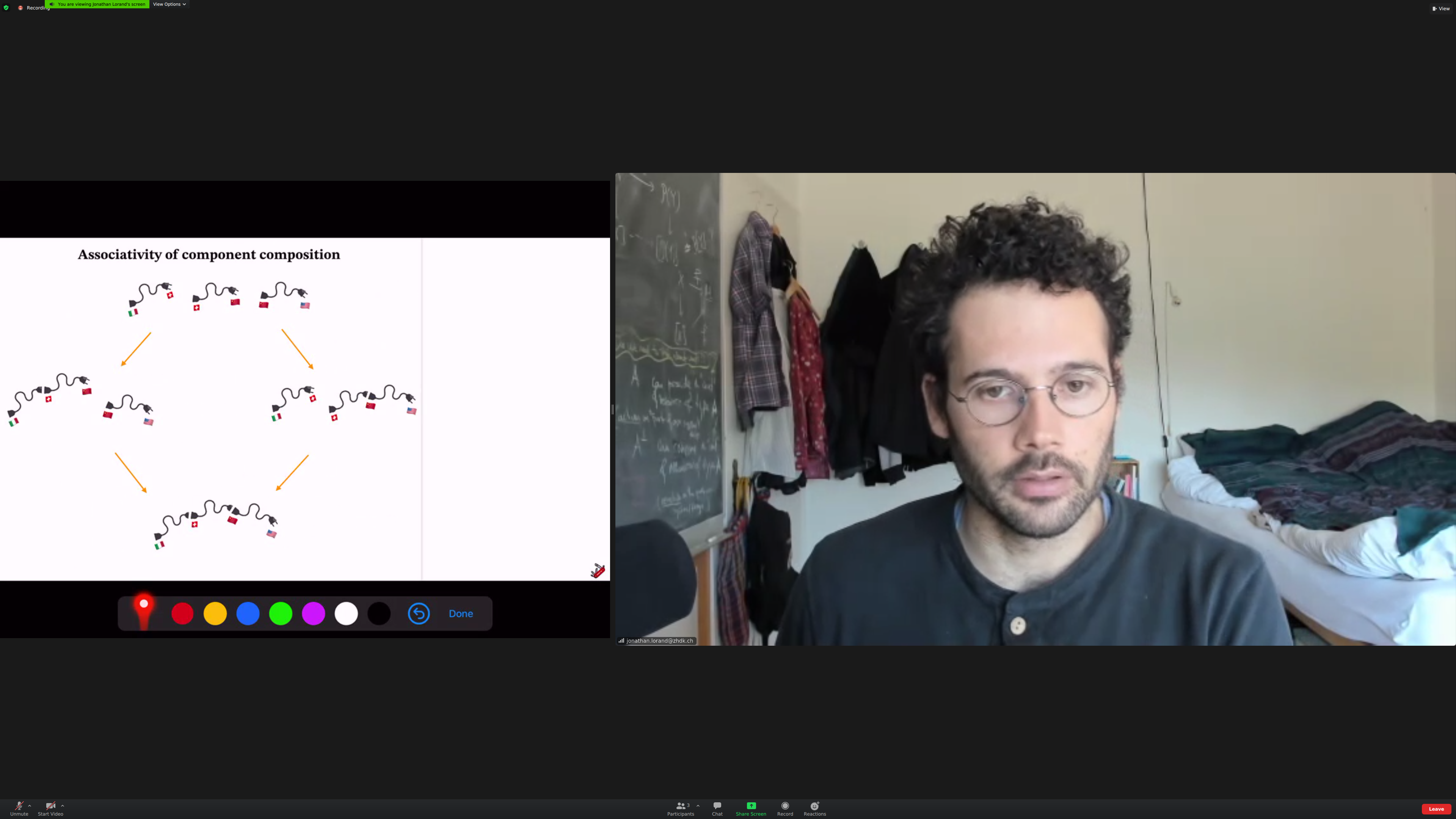Identity morphisms: morphisms that do nothing. Like a zero in addition.

Identity morphism for functions: the identity function.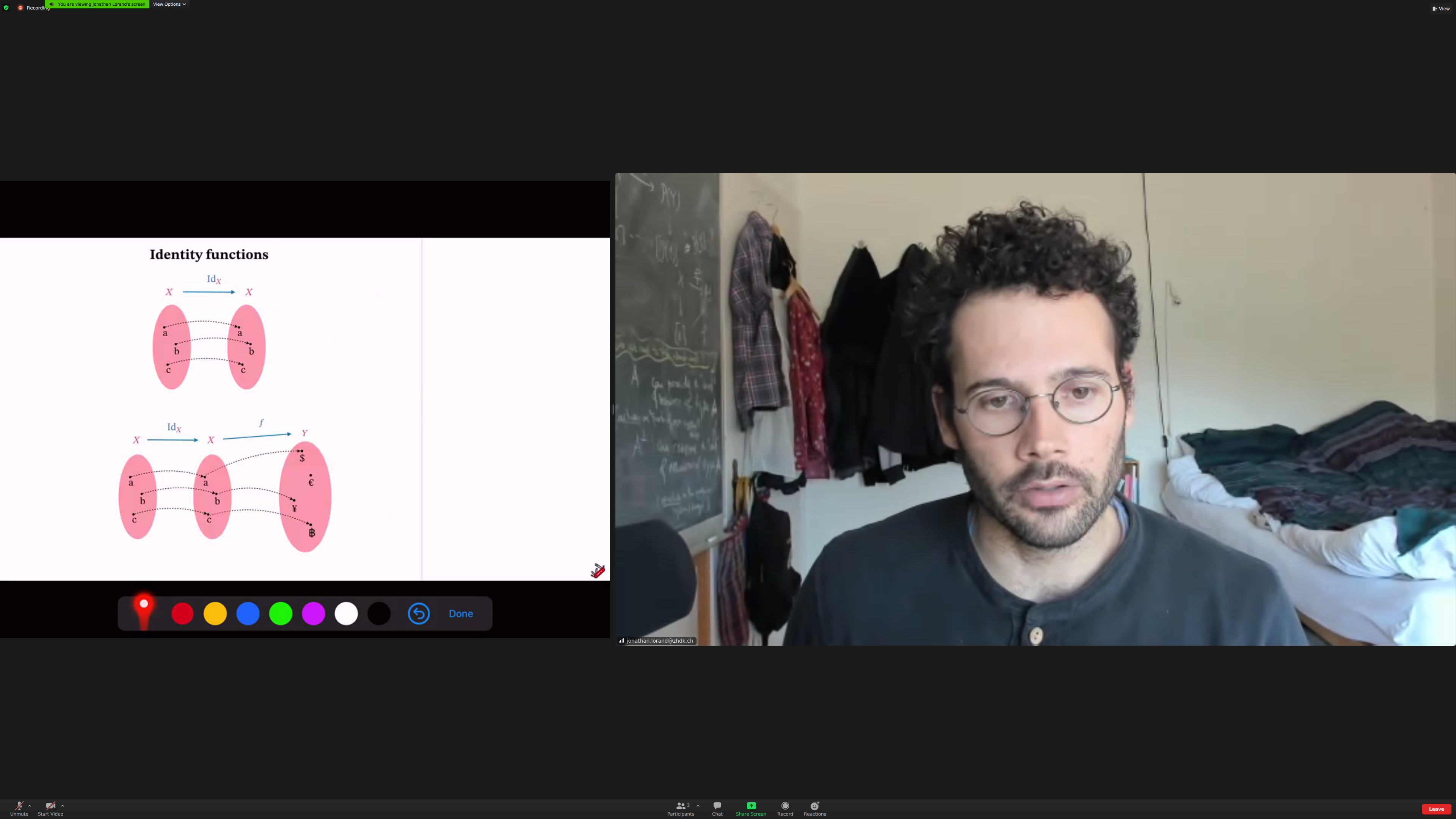A converter that converts to the same electrical standard is just an extension that can become a converter of any type with one additional composition.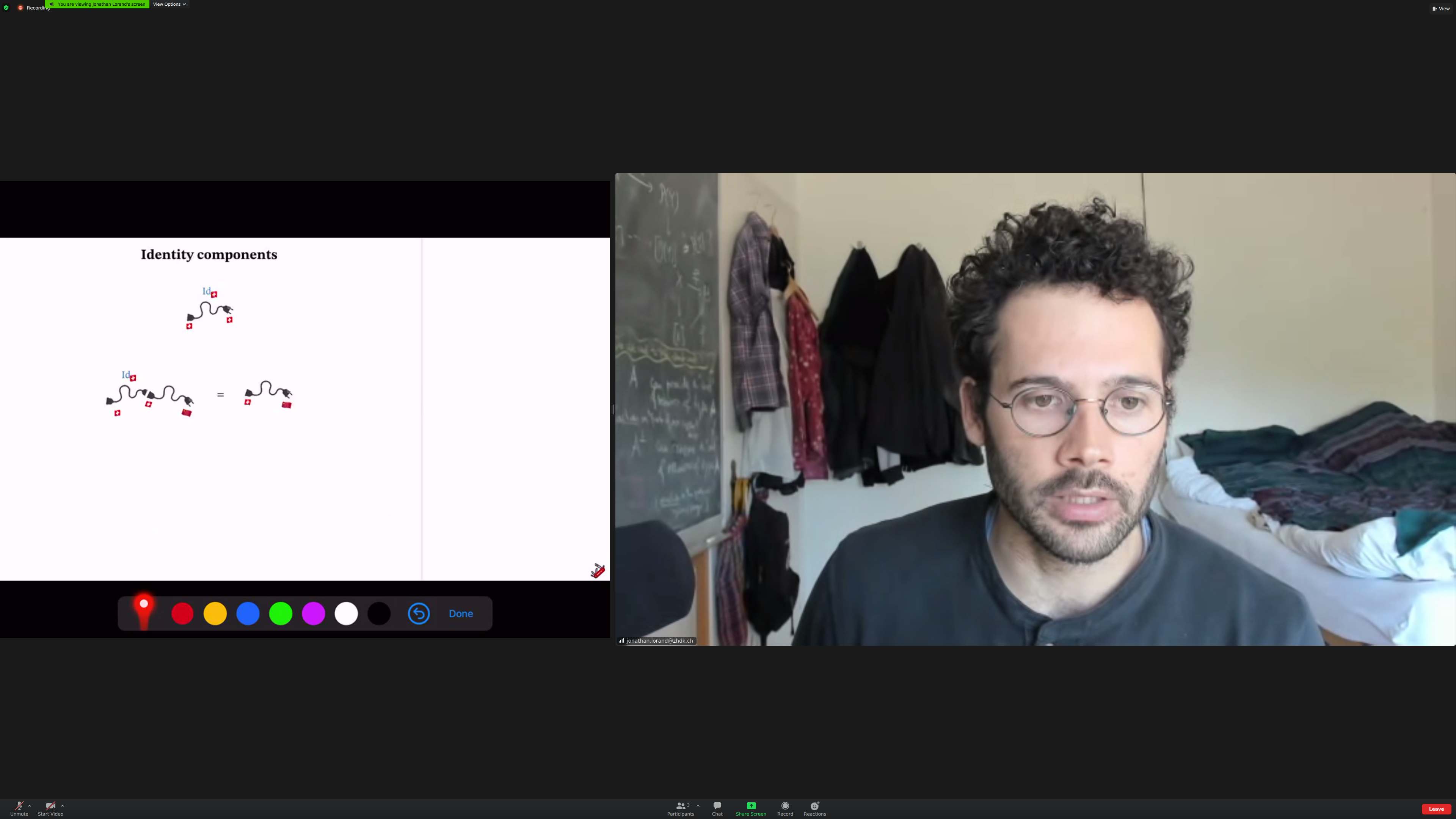[[identity journeys]] (aside: I call these [[excursions]])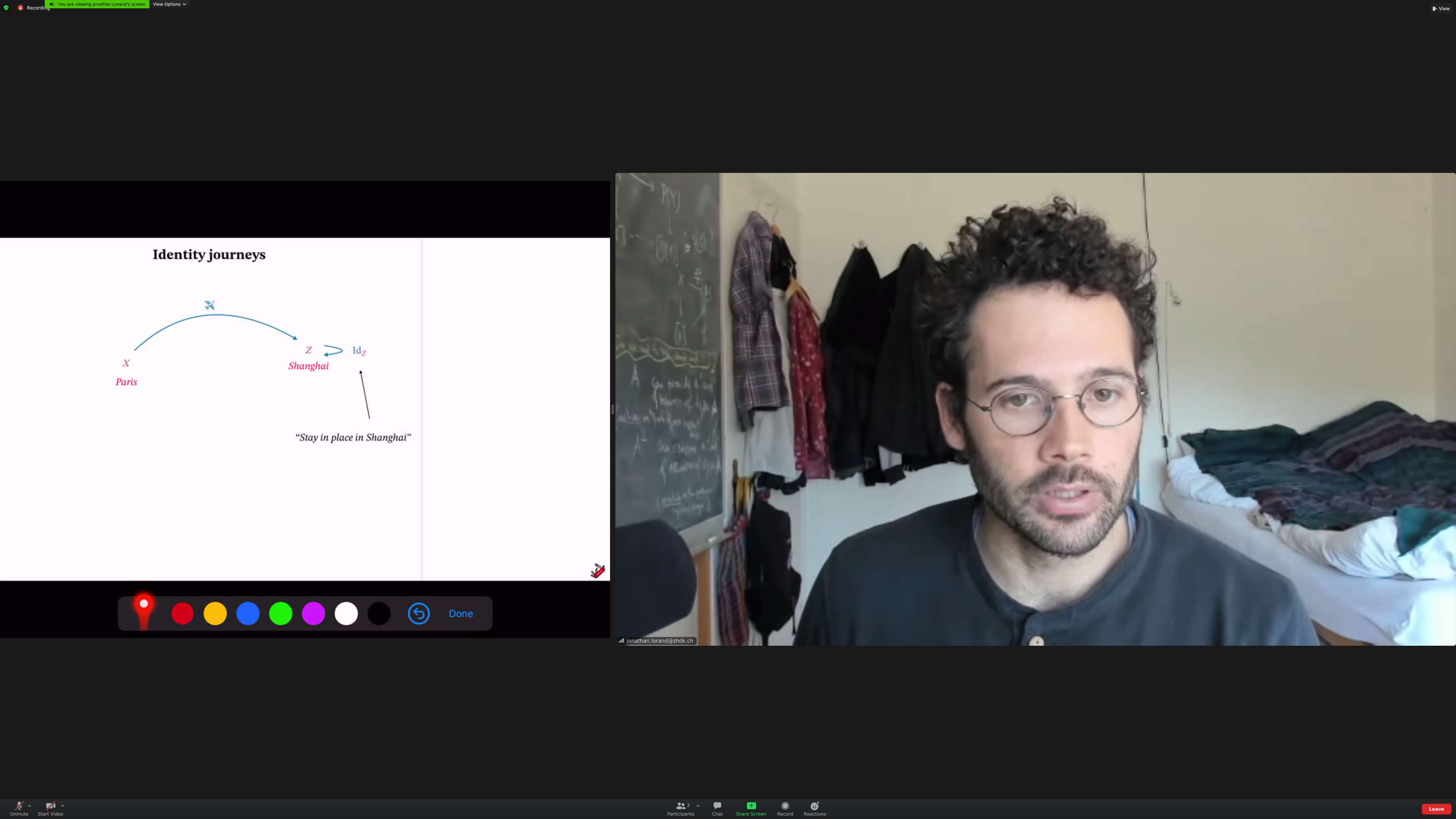Mathematical notion of a category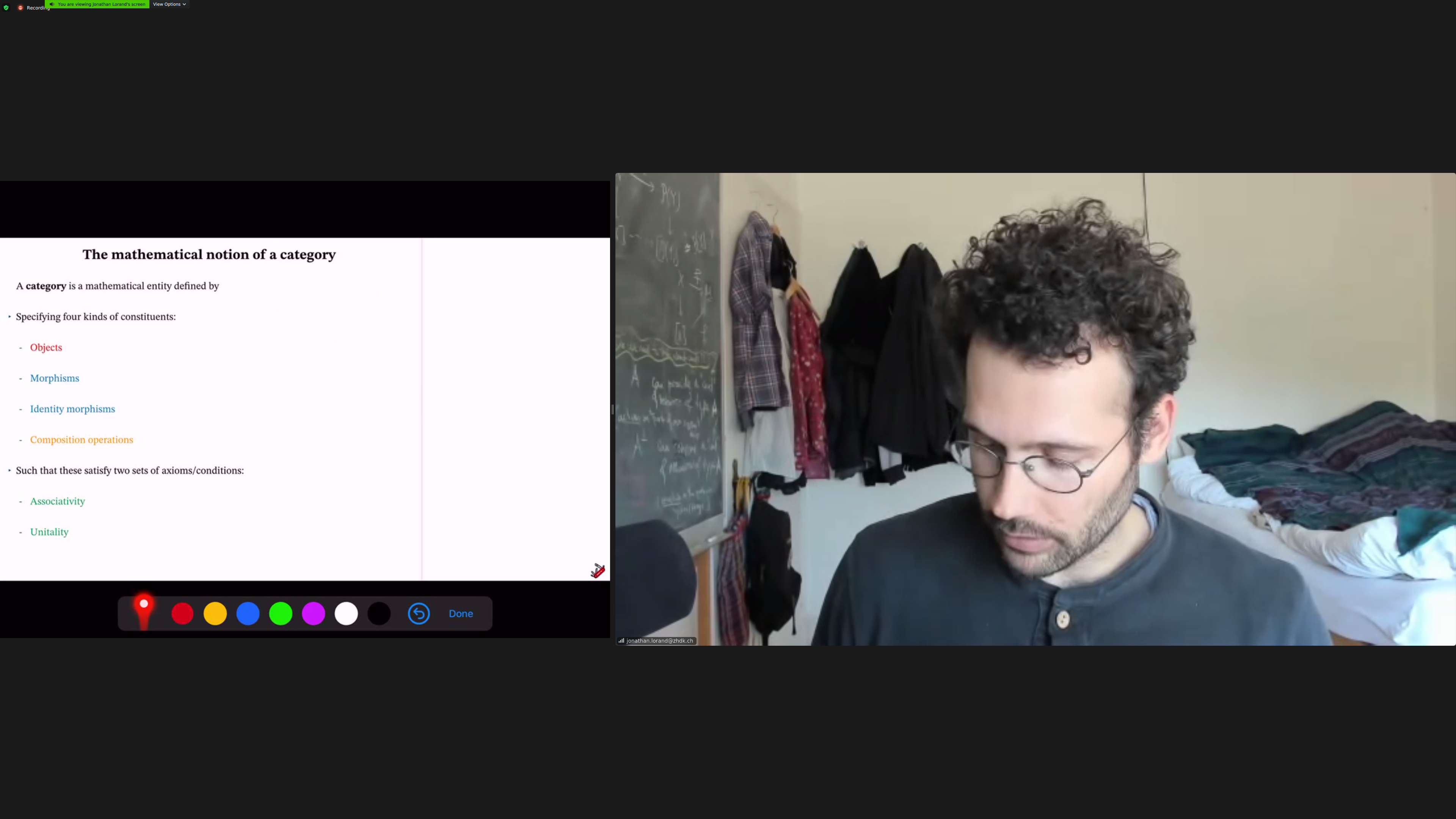Aside: I think most concepts had been introduced before except one?

"F is a morphism from X to Y":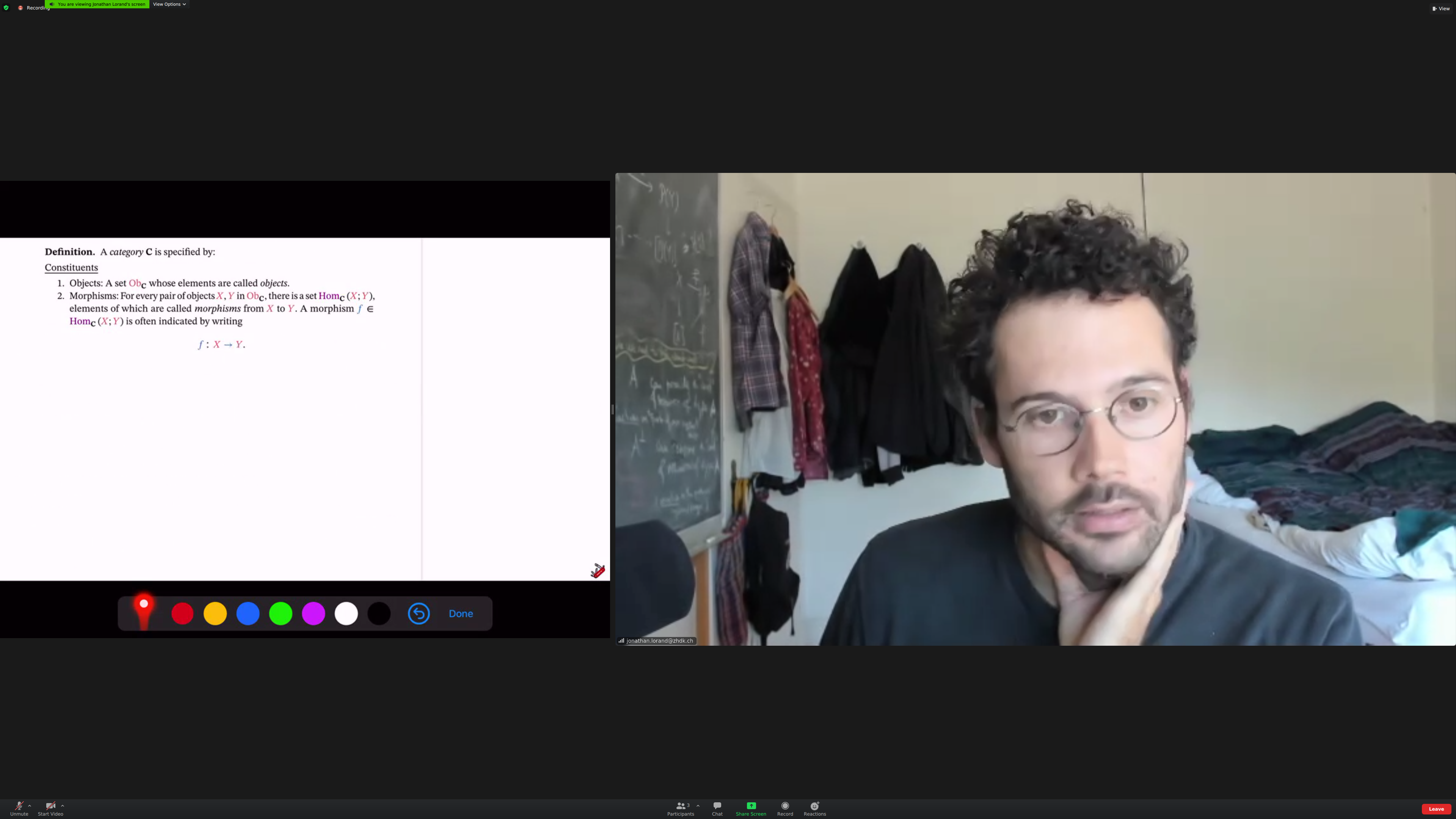Identity morphisms: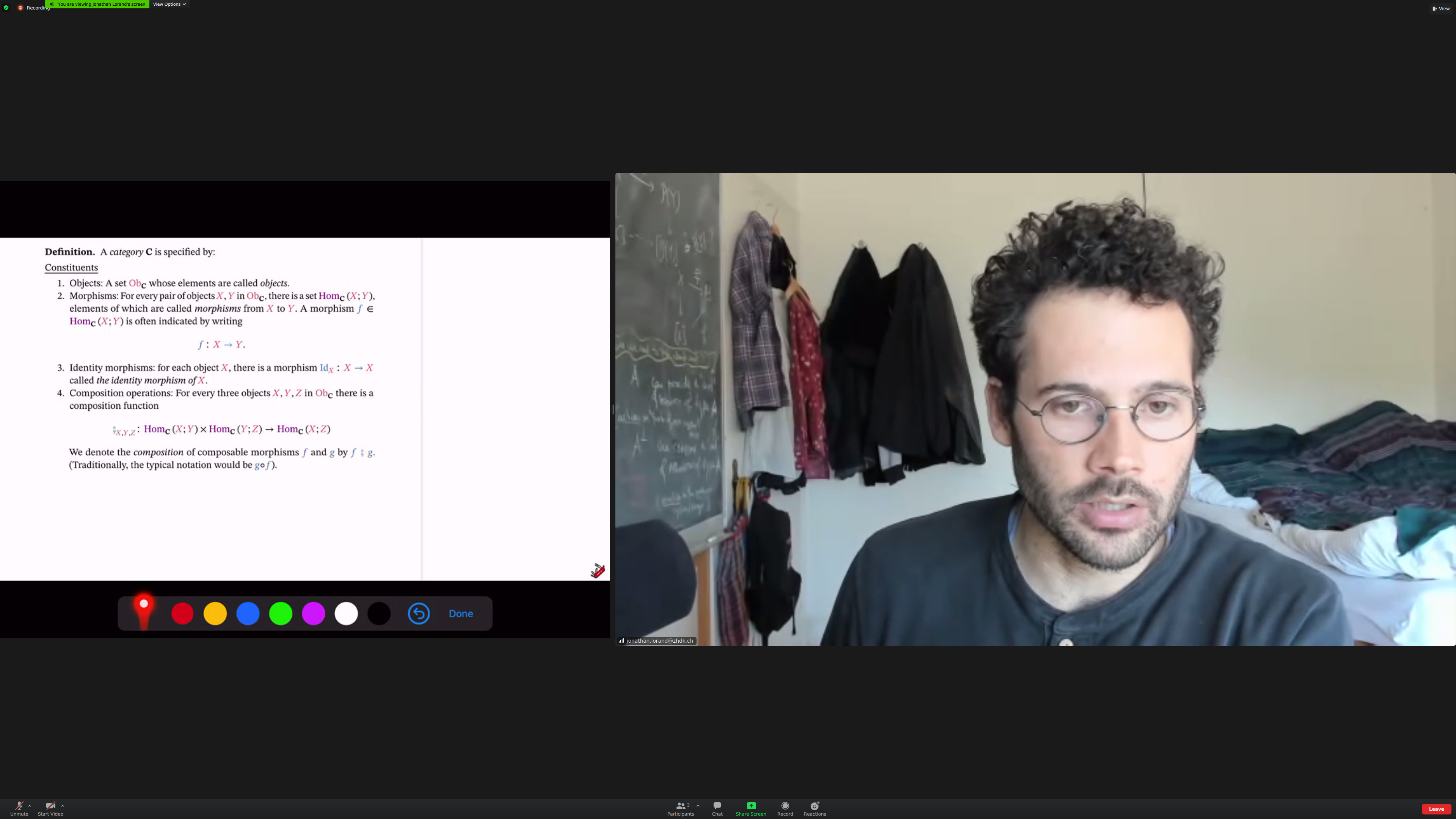Composition: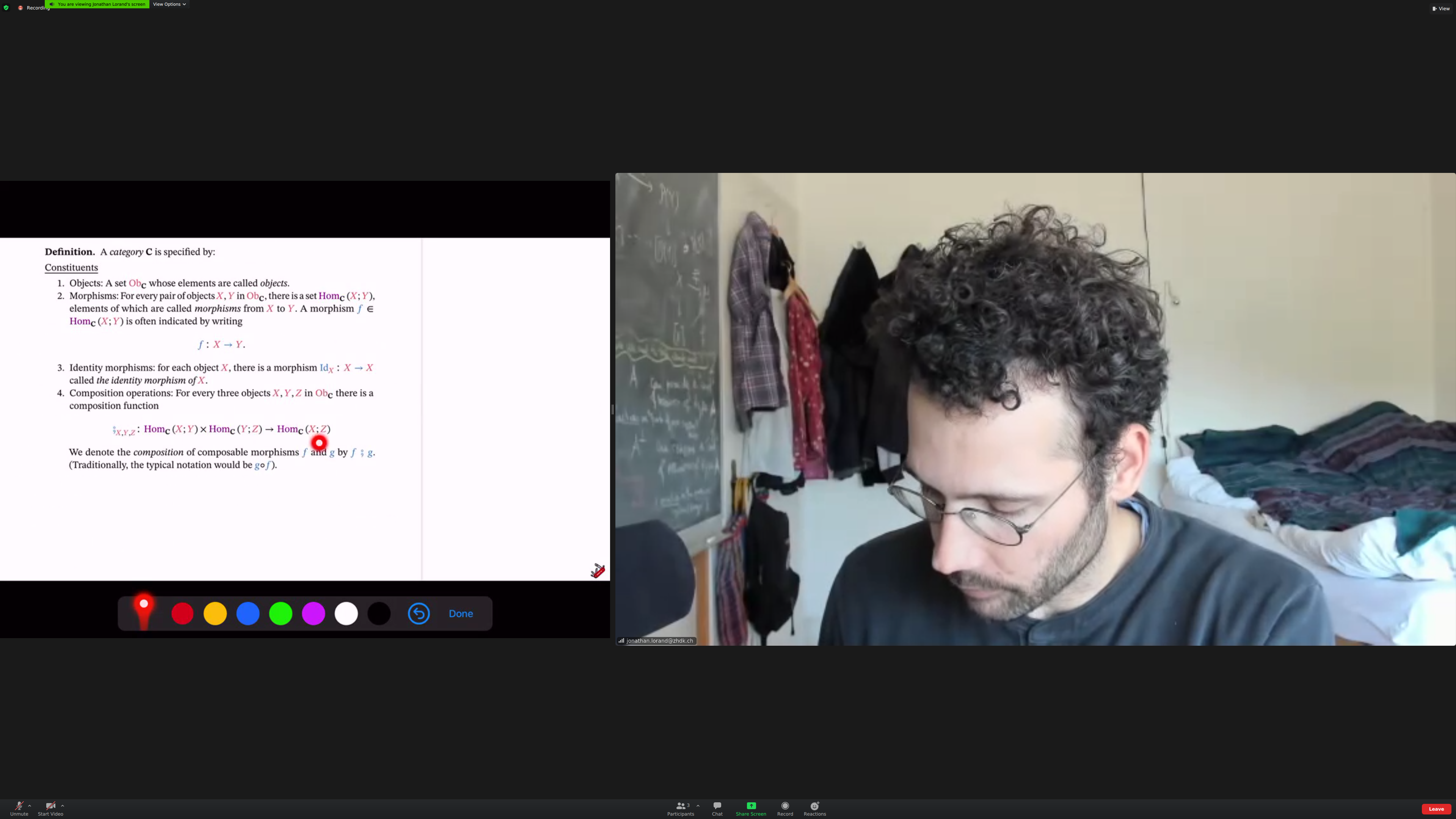Unitality: identity "works" (I think this was the concept I thought hadn't been defined previously):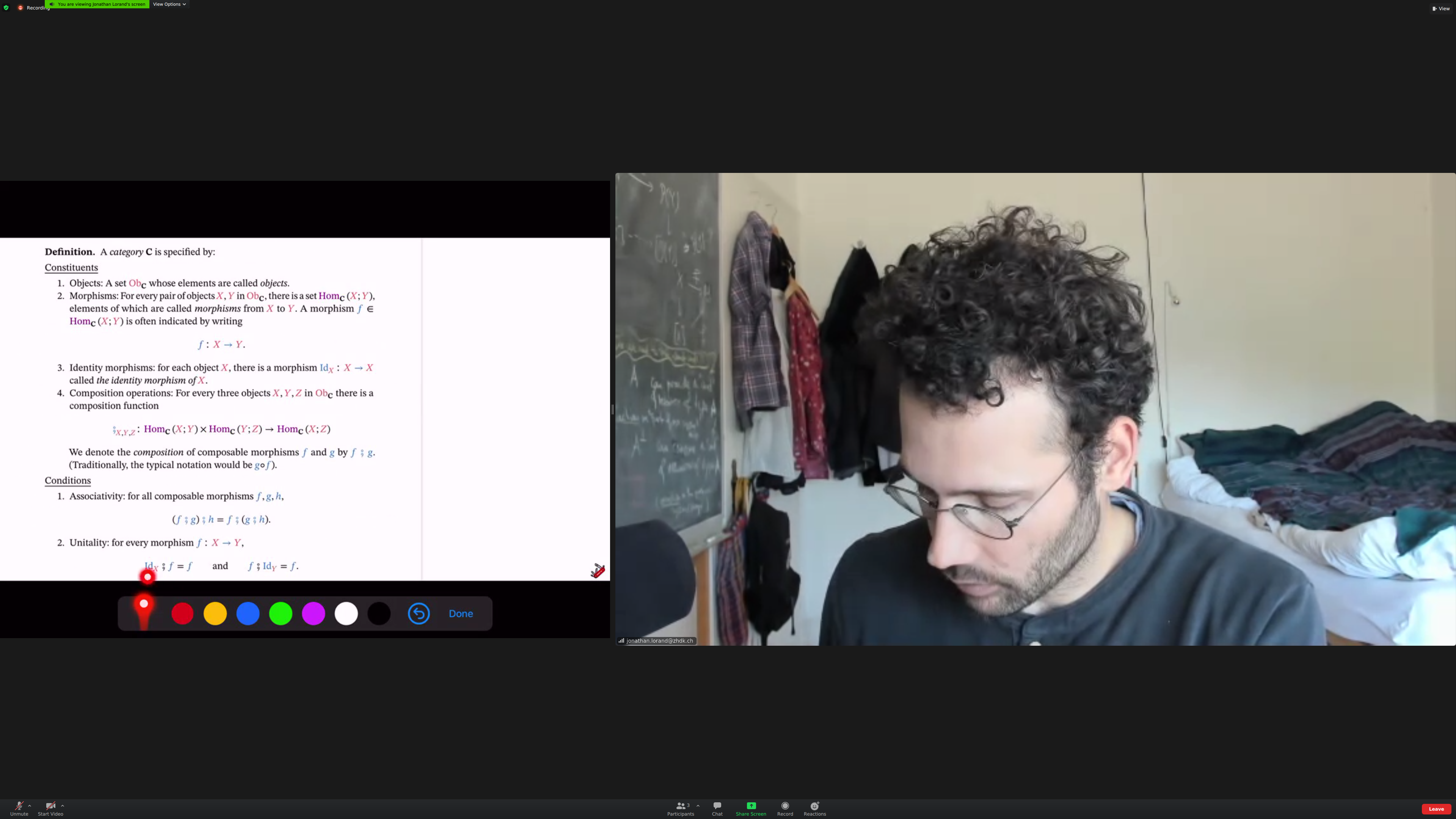The [[category of sets and functions]] plays a central role in category: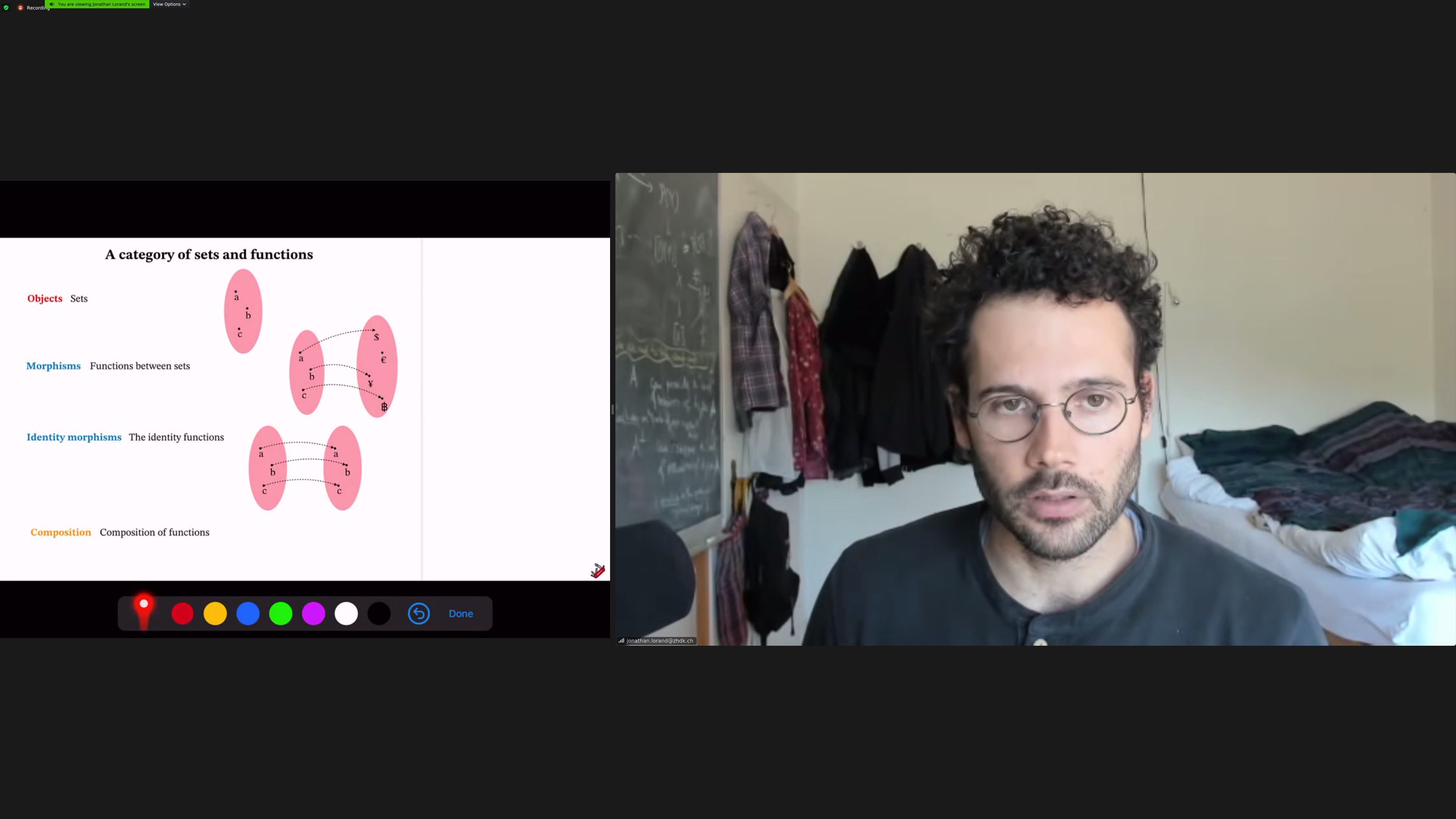The [[category of plugs and chords]]: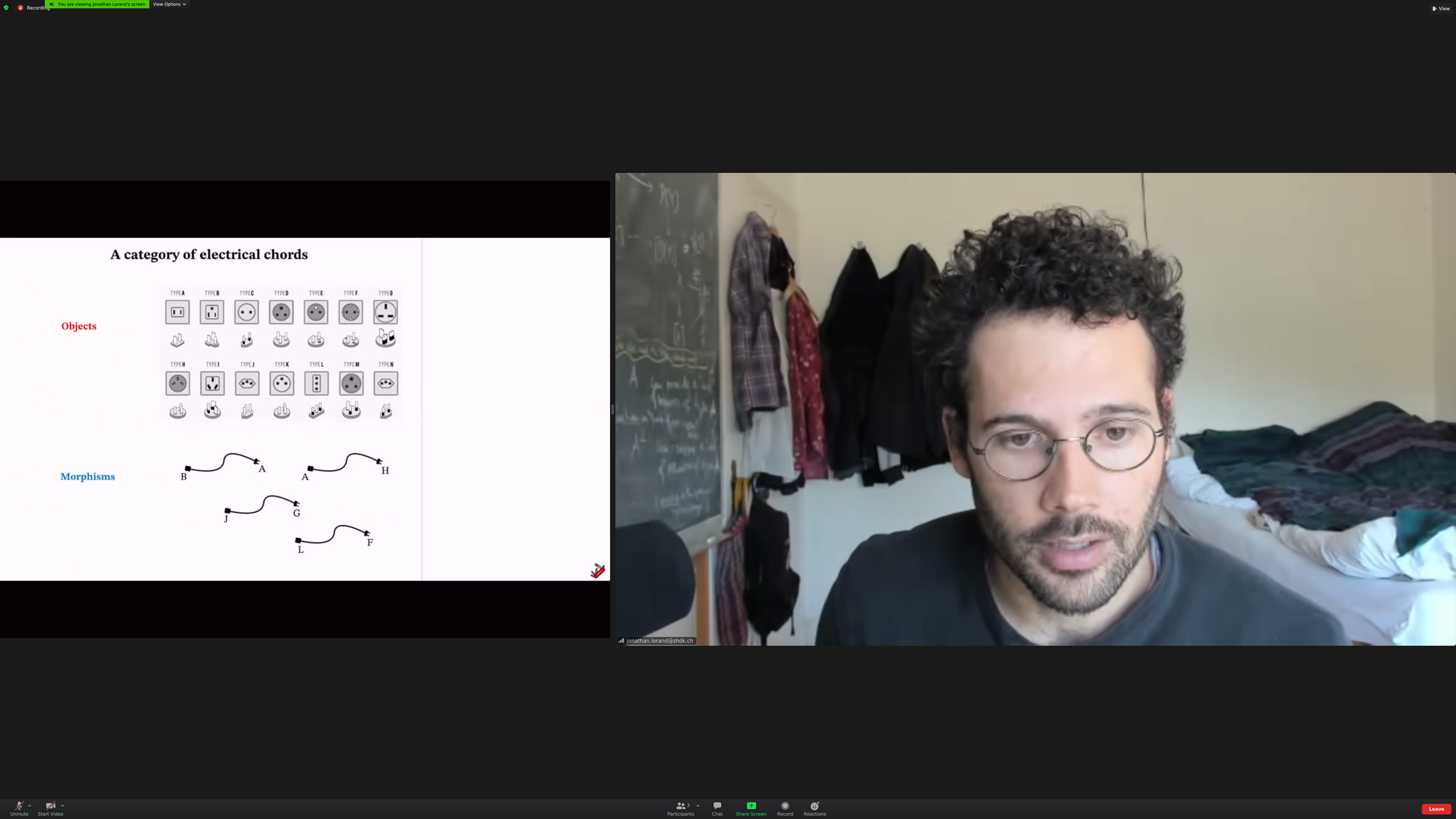The [[category of journeys]]: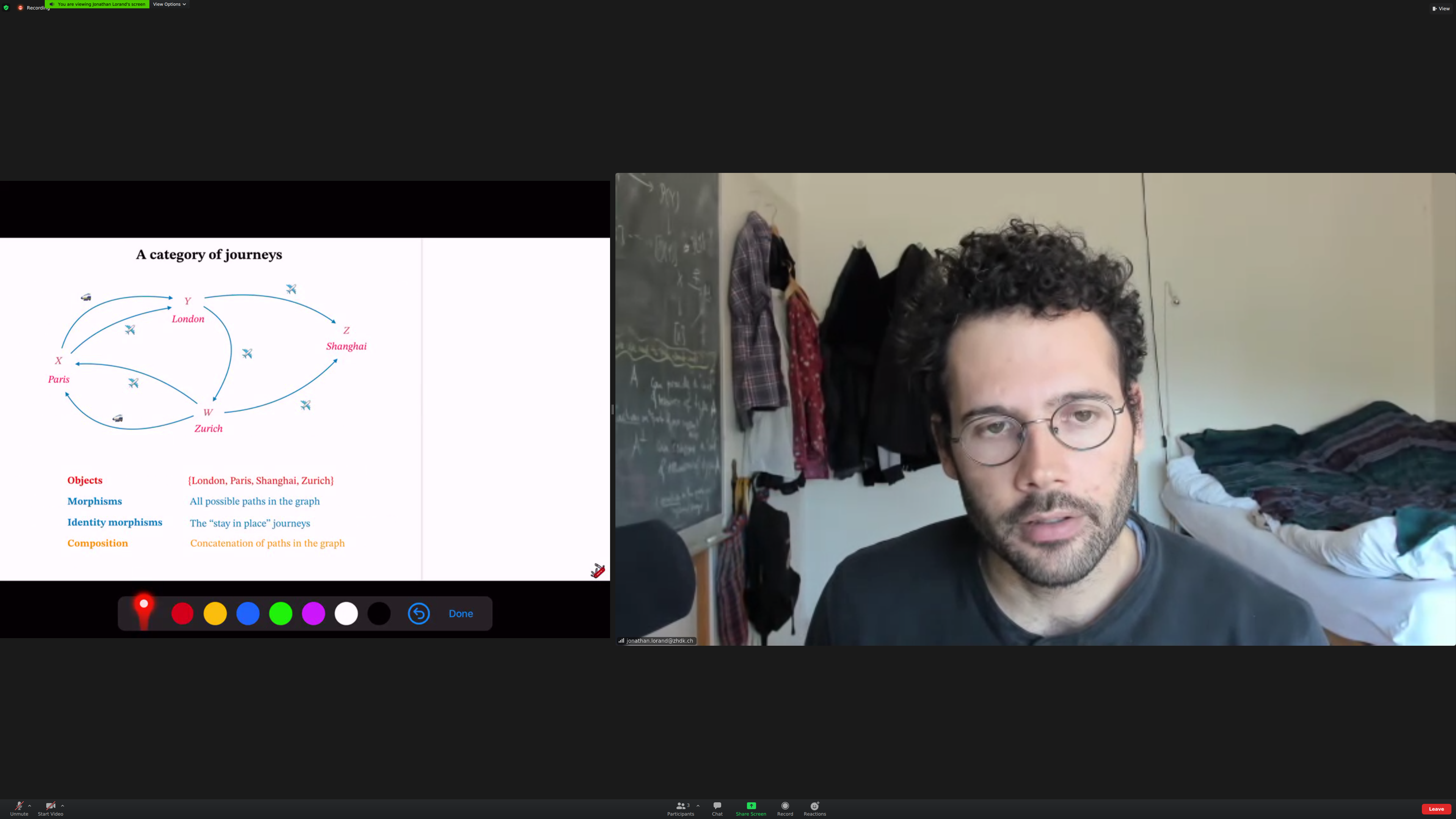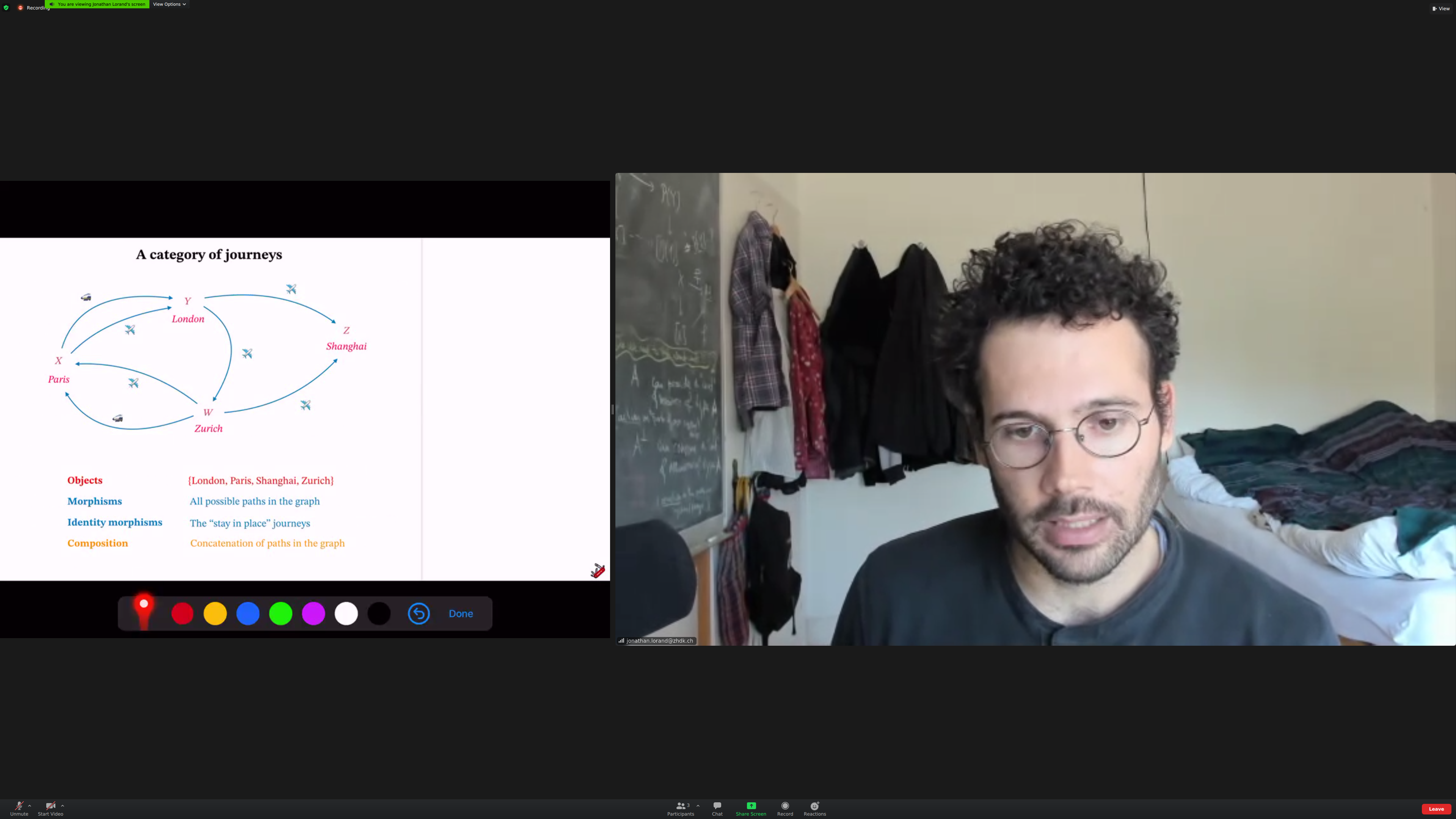(Aside: some screenshots might be duplicate, they are likely from two moments that felt significant)

Any directed graph generates a category.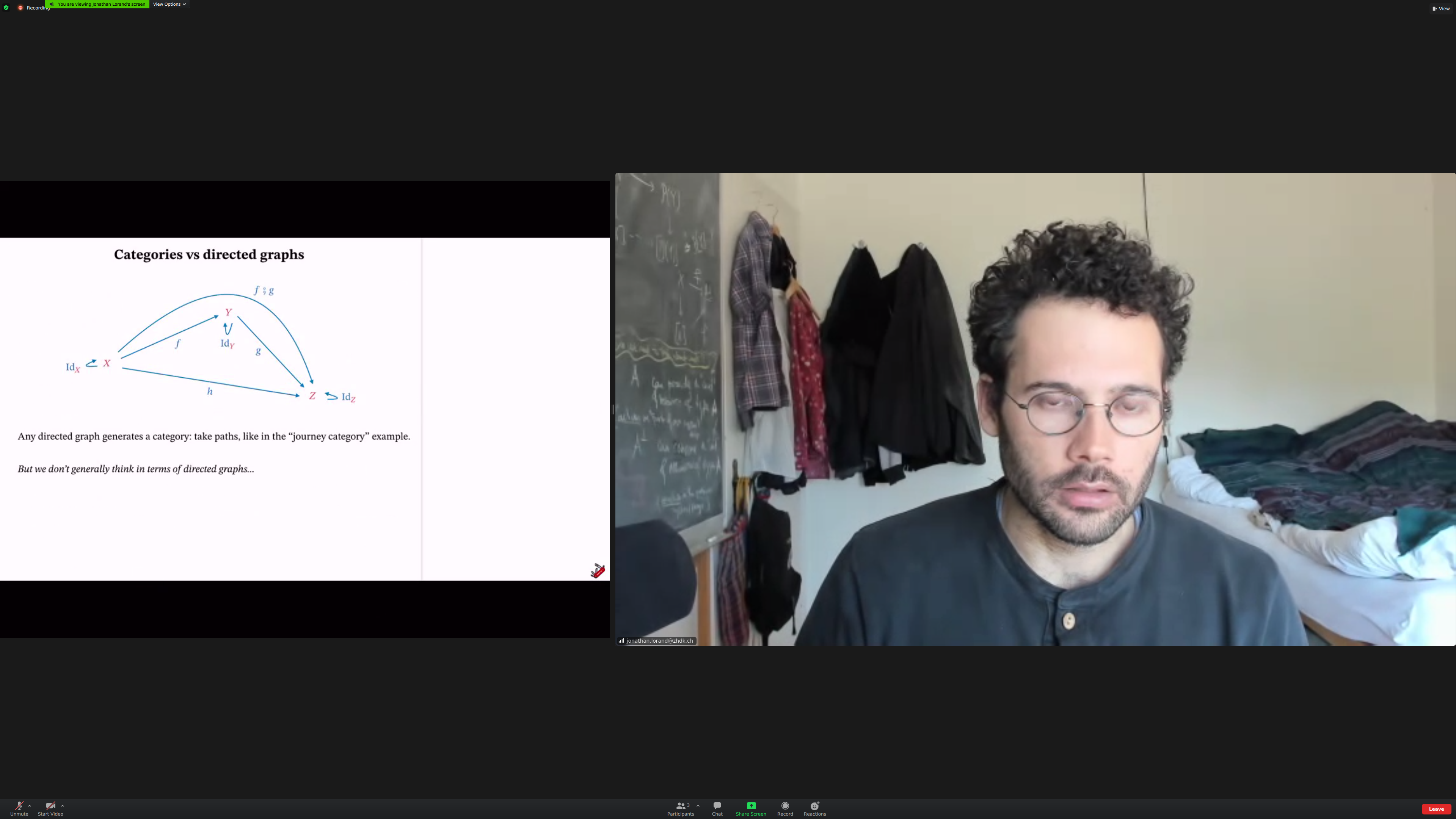(Aside: a directed graph generates dependency trees.)

But categories are [[richer]] than [[directed graphs]], so they aren't used that much as such.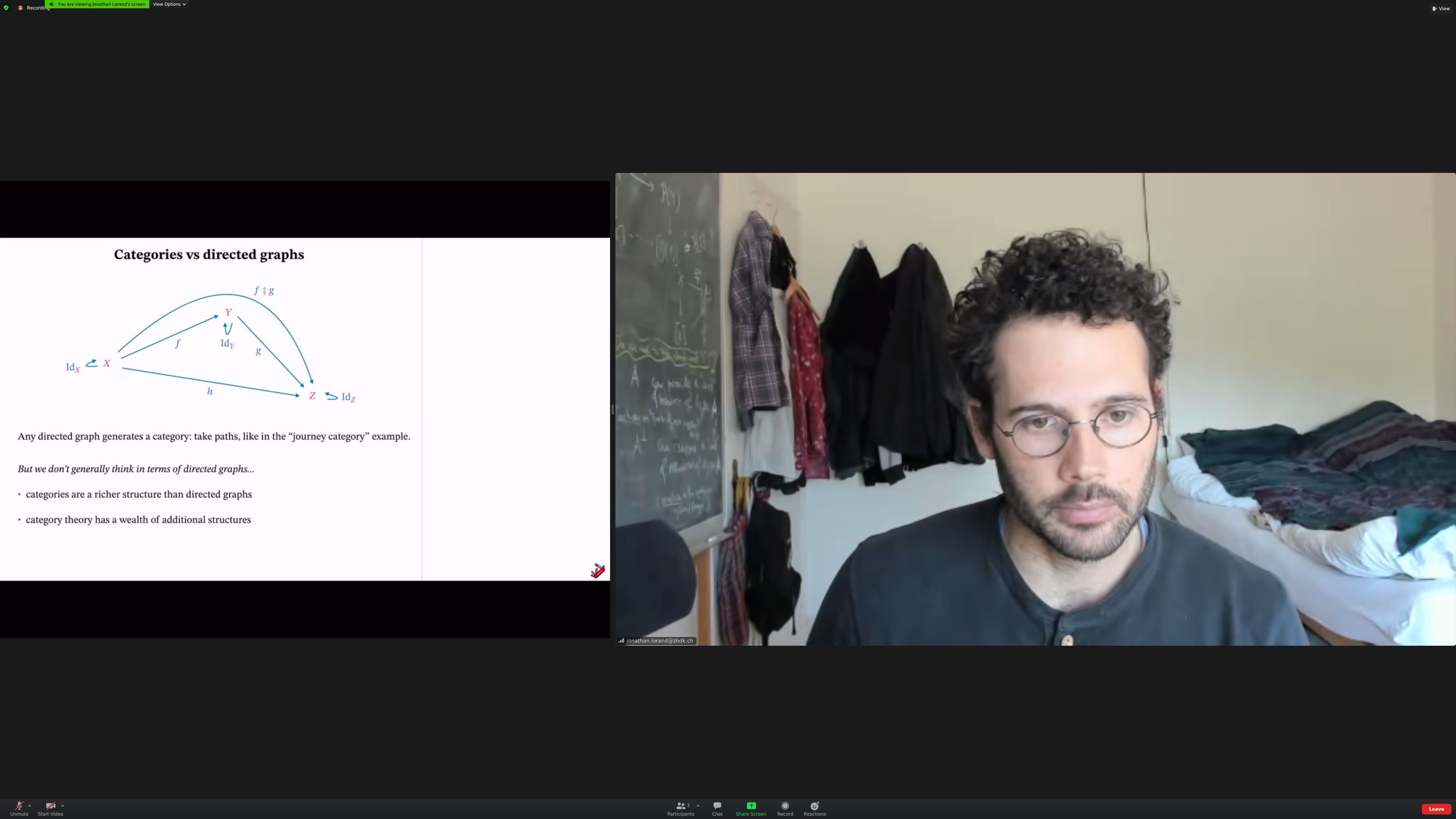Category theory is interested in [[relations between morphisms]].if f;g is equal to h, this diagram [[commutes]].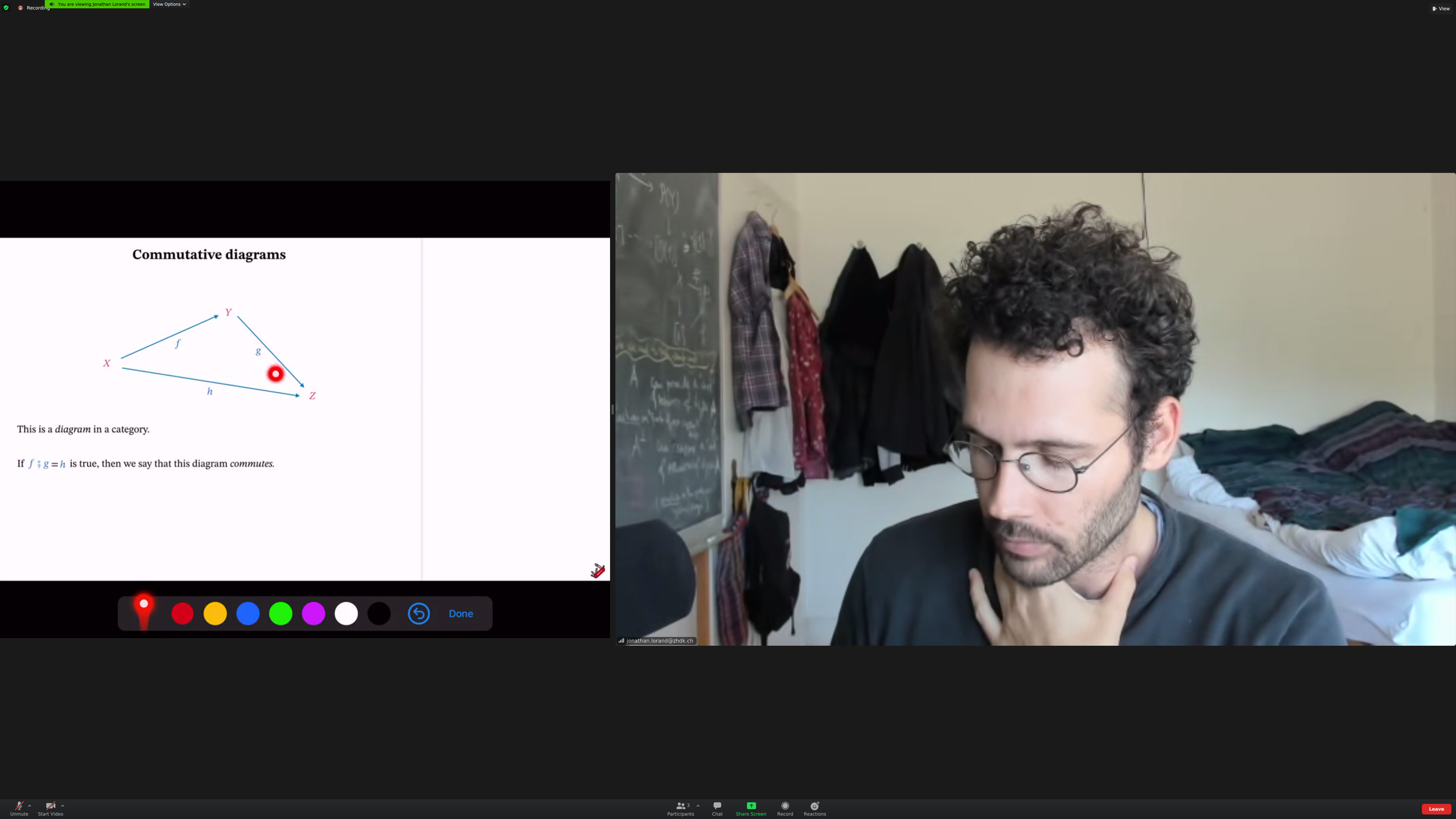Aside: when you say 'if you compose' something, it'd be nice to have the right notation for the composition on screen.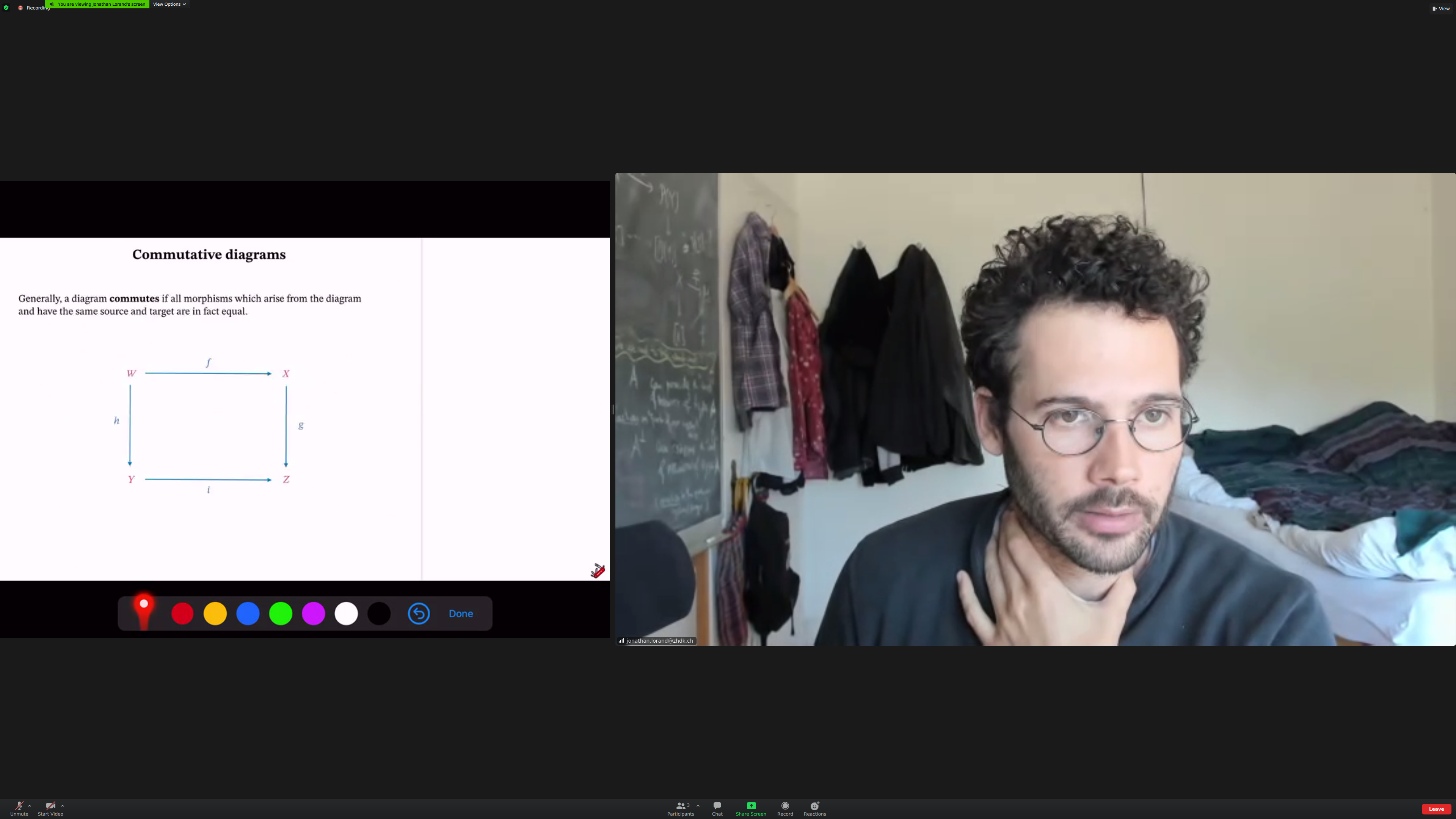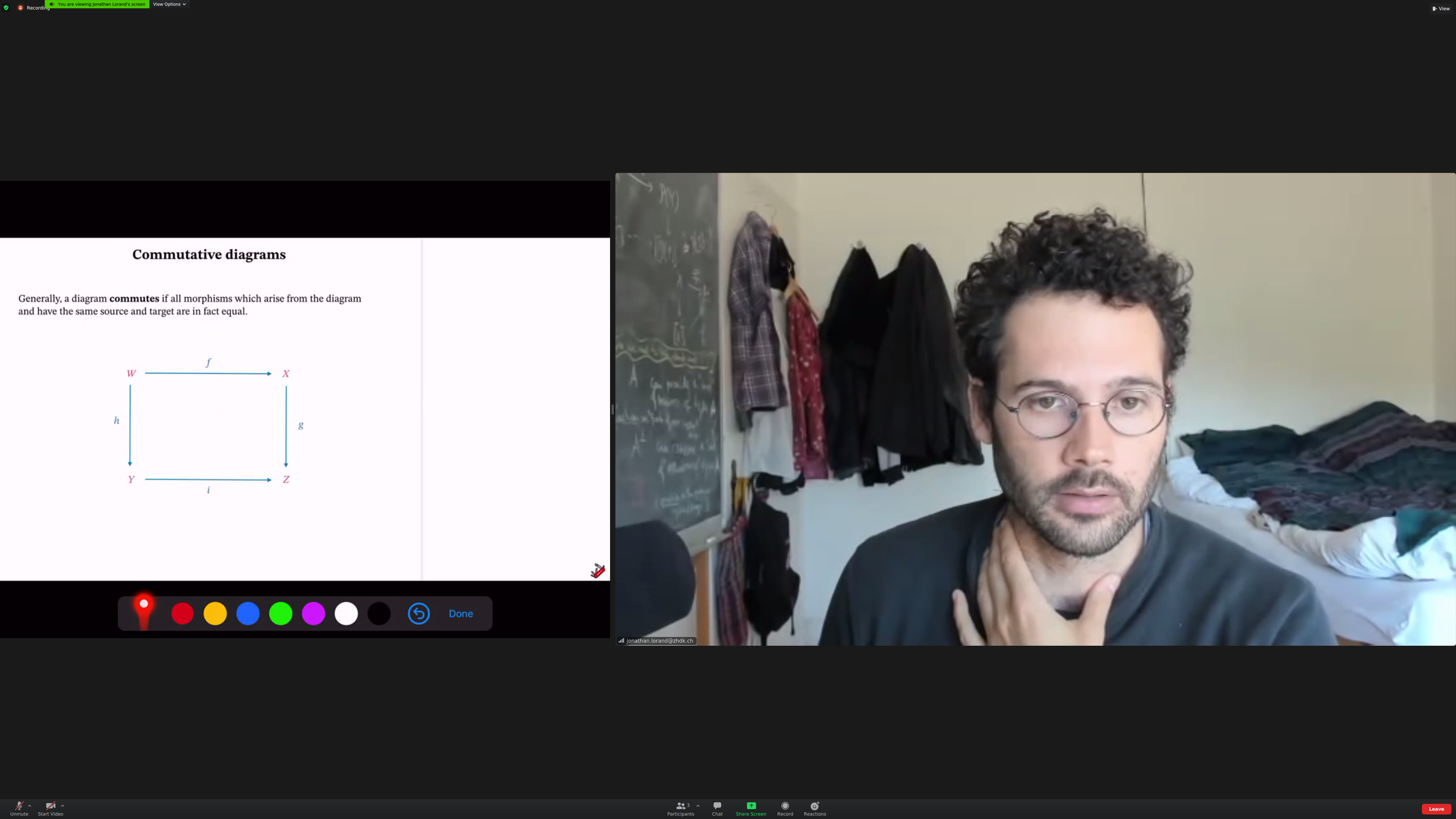Sameness: [[isomorphism]]. Identity is equal to roundtrip.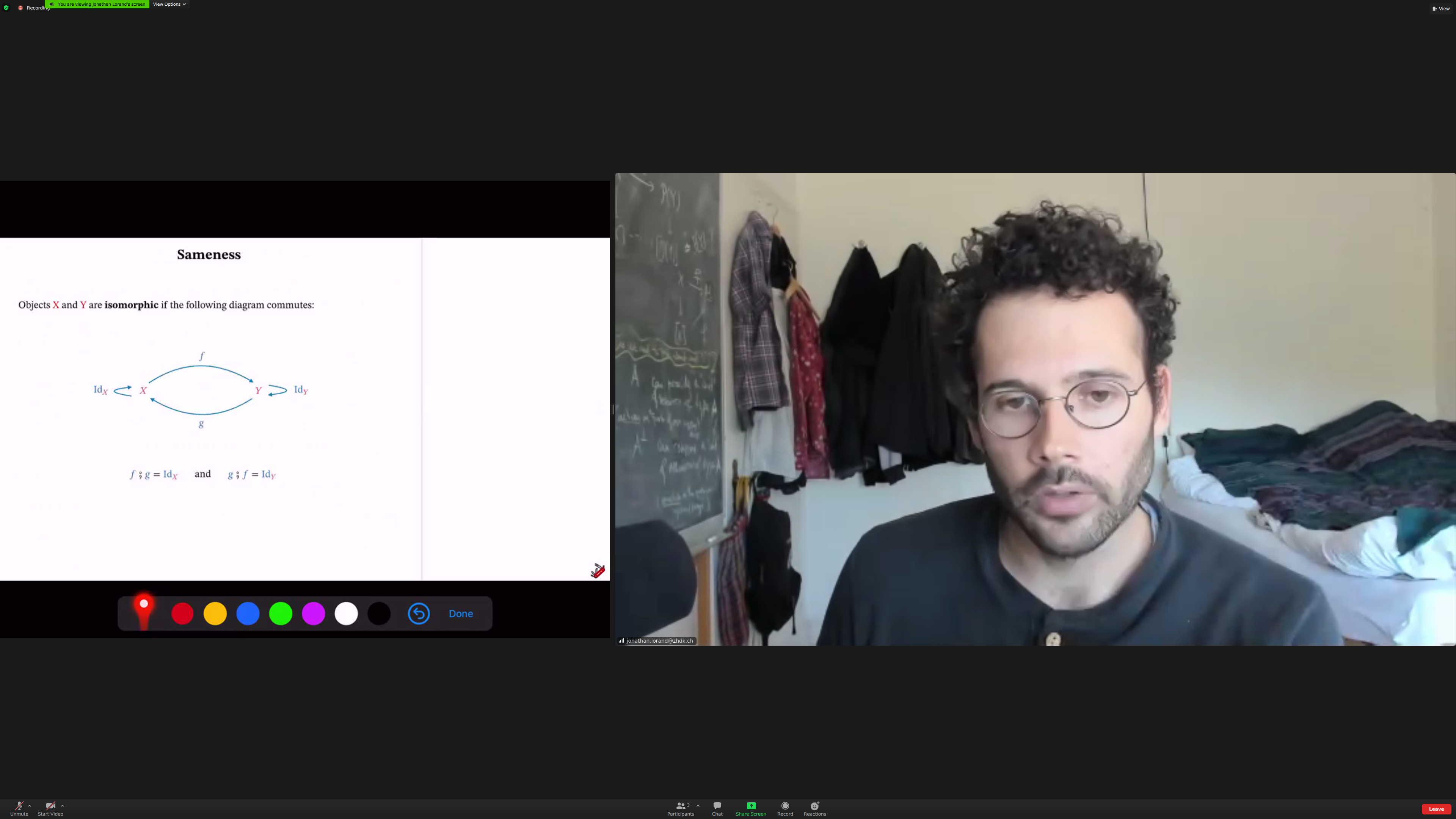(in the category of sets/functions, an isomorphism is equivalent to a [[bijection]]. in the category of topological spaces and continuous maps, it is a [[homeomorphism]])

A context.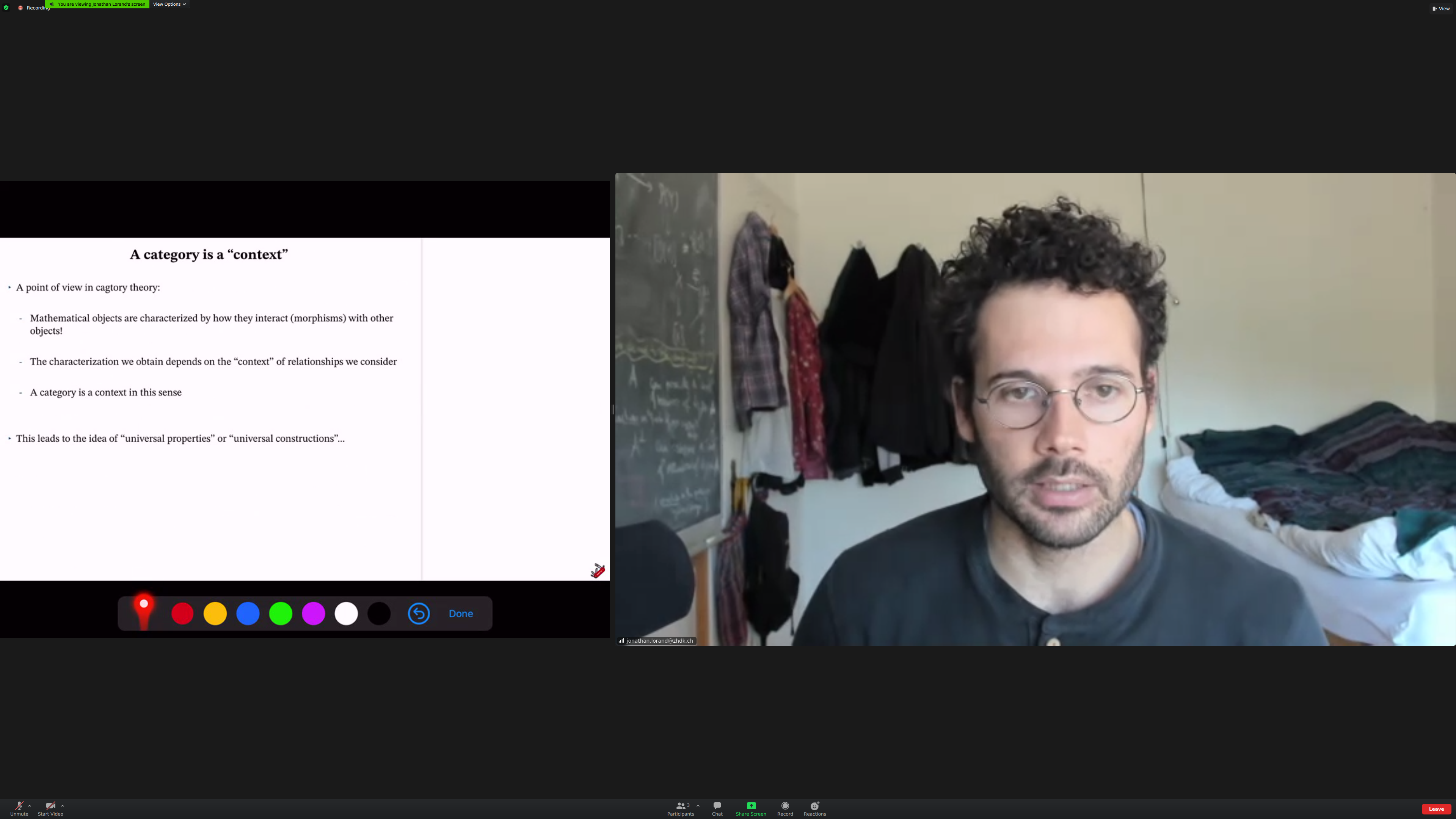(Aside: I needed more time in this slide).

Contexts leads to [[universal constructions]] and [[universal properties]].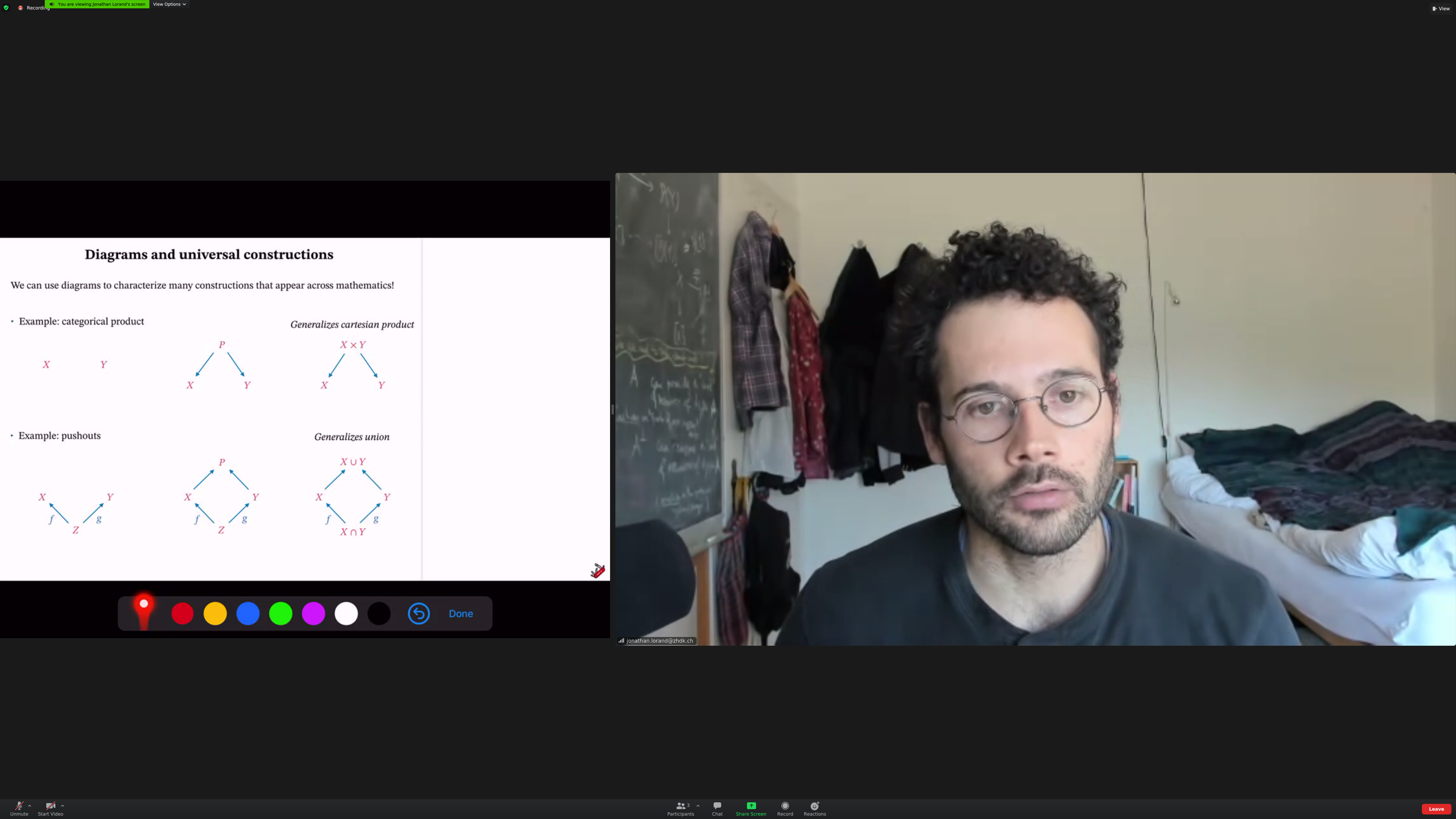Example: [[categorical product]].

You start with objects, no morphisms. From the left diagram, you construct one with [[morphisms]]. In this one, we generalize cartesian product.[[pushouts]] Using the information given by f and g, you [[integrate]] two objects (check).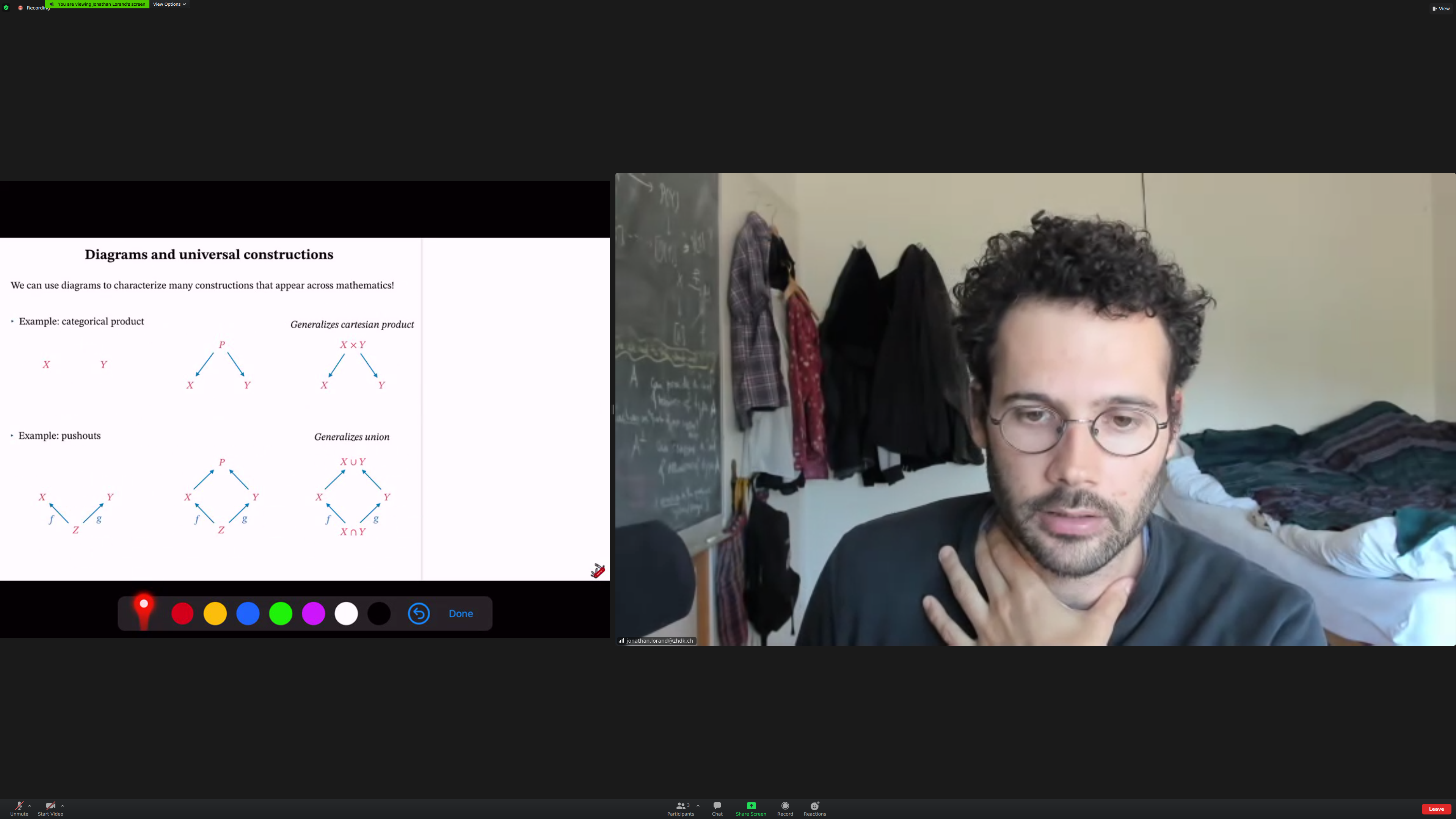Additional structures: [[monoidal products]]. A way to compose objects and morphisms "in parallel".

(Aside: it would be nice to have original and post application of composition side by side or top to bottom).

(Aside: missing screenshot here, find in Pictures)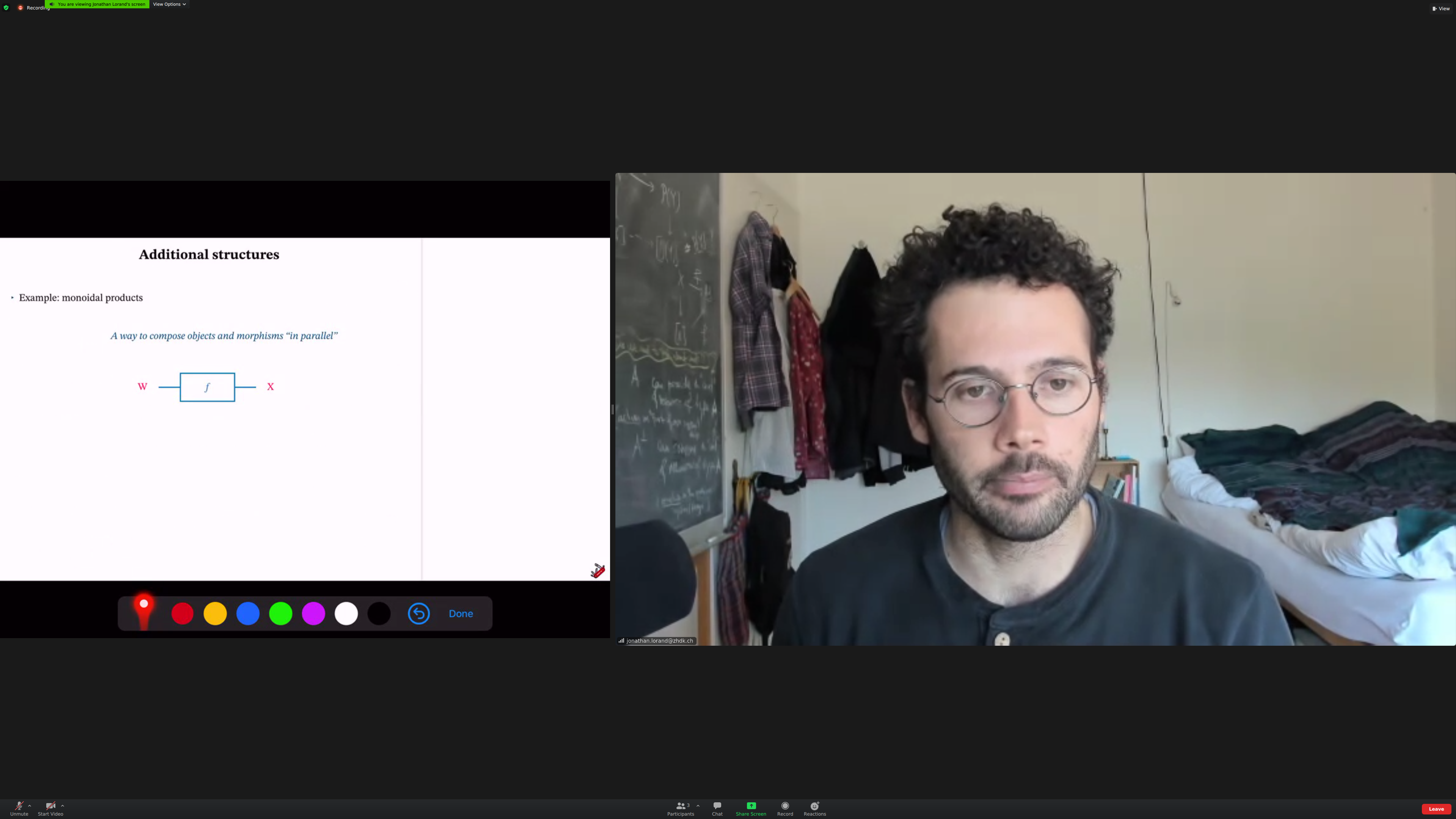📖 stoa (open document) at doc.anagora.org/category-theory-basics
⥱ context
⥅ related node [[2021 05 30]]
⥅ related node [[category theory]]
⥅ related node [[category theory]]
⥅ related node [[class]]
⥅ related node [[context]]
⥅ related node [[target]]